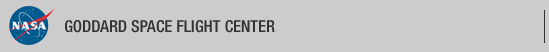+ Visit NASA.gov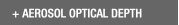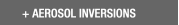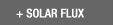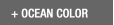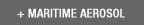Web Site Feature AERONET Data Synergy Tool - Access Earth Science data sets for AERONET sites+Home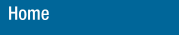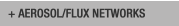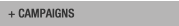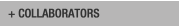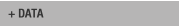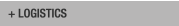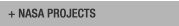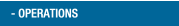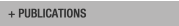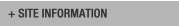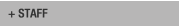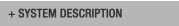AERONET DATA ACCESS

AERONET Site Lists (V3)

+ All Lists# Report of DCP transmissions

 Status Legend

AERONET No 6 [1317E33A] - 28 msg rcvd (12447 b) site : GSFC (Last Tx is before schedule by 0 min 3 sec
28 messages stepped outside their assigned time window)
AERONET No 26 [1316E1C0] - 28 msg rcvd (17341 b) site : GSFC (Last Tx is late by 0 min 1 sec)
AERONET No 28 [13101F58] - 28 msg rcvd (19569 b) site : White_Sands_HELSTF (Last Tx is precisely on time)
AERONET No 59 [131A03AE] - 28 msg rcvd (4124 b) site : Sevilleta (Last Tx is precisely on time)
AERONET No 134  - 7 msg rcvd (539 b) site : GSFC (Last Tx is before schedule by 0 min 3 sec
7 messages stepped outside their assigned time window)
AERONET No 163  - 28 msg rcvd (17275 b) site : UCSB (Last Tx is precisely on time)
AERONET No 196 [1319A458] - 28 msg rcvd (21767 b) site : Rio_Branco (Last Tx is precisely on time)
AERONET No 306 [131A40A4] - 28 msg rcvd (6210 b) site : Easton-MDE (Last Tx is precisely on time , 43b)
AERONET No 307 [1318152C] - 28 msg rcvd (14513 b) site : Ames (Last Tx is late by 0 min 1 sec , 4b)
AERONET No 317 [131982B4] - 28 msg rcvd (17146 b) site : Tudor_Hill (Last Tx is precisely on time)
AERONET No 319 [131820B6] - 28 msg rcvd (7202 b) site : Trelew (Last Tx is late by 0 min 1 sec 243s)
AERONET No 330 [131481D2] - 28 msg rcvd (23036 b) site : GSFC (Last Tx is late by 0 min 1 sec)
AERONET No 365 [131A53D2] - 27 msg rcvd (13850 b) site : Ji_Parana_SE (Last Tx is precisely on time , 24b)
AERONET No 374 [131713BE] - 28 msg rcvd (23348 b) site : TABLE_MOUNTAIN_CA (Last Tx is precisely on time)
AERONET No 409 [131AA356] - 27 msg rcvd (9998 b) site : Mingo (Last Tx is precisely on time)
AERONET No 601 [1319E752] - 25 msg rcvd (2800 b) site : CCNY ()
AERONET No 624 [131AE05C] - 28 msg rcvd (3108 b) site : CUIABA-MIRANDA ()
AERONET No 635 [1319D2C8] - 28 msg rcvd (14744 b) site : Alta_Floresta (Last Tx is precisely on time)
AERONET No 813 [1310118A] - 28 msg rcvd (28468 b) site : Yuma (Last Tx is precisely on time 4s, 8b)

# Aeronet No 6 [1317E33A] (GSFC)

Last Tx is before schedule by 0 min 3 sec
28 messages stepped outside their assigned time window

11:12:2019,10:41:47 (UTC) 'G' (DCP total: 87) Cimel : 6 time corr. = -27 sec (DCP Volt = 13.00 Lat= 38.993 Long= -76.841(0.1 km from GSFC))
11:12:2019,11:41:47 (UTC) 'G' (DCP total: 87) Cimel : 6 time corr. = -27 sec (DCP Volt = 13.00 Lat= 38.993 Long= -76.841(0.1 km from GSFC))
11:12:2019,12:41:47 (UTC) 'G' (DCP total: 87) Cimel : 6 time corr. = -26 sec (DCP Volt = 13.00 Lat= 38.992 Long= -76.841(0.1 km from GSFC))
11:12:2019,13:41:47 (UTC) 'G' (DCP total: 263) Cimel : 6 time corr. = -27 sec (DCP Volt = 13.00 Lat= 38.993 Long= -76.840(0.0 km from GSFC)) staD nsu stah stah
11:12:2019,22:41:47 (UTC) 'G' (DCP total: 777) Cimel : 6 time corr. = -28 sec (DCP Volt = 13.00 Lat= 38.993 Long= -76.840(0.0 km from GSFC)) nsu staD nsu staD nsu staD nsu staD nsu staD nsu staD
11:12:2019,23:41:47 (UTC) 'G' (DCP total: 87) Cimel : 6 time corr. = -28 sec (DCP Volt = 13.00 Lat= 38.993 Long= -76.840(0.0 km from GSFC))
12:12:2019,00:41:47 (UTC) 'G' (DCP total: 87) Cimel : 6 time corr. = -28 sec (DCP Volt = 13.00 Lat= 38.993 Long= -76.840(0.0 km from GSFC))
12:12:2019,01:41:47 (UTC) 'G' (DCP total: 87) Cimel : 6 time corr. = -28 sec (DCP Volt = 13.00 Lat= 38.993 Long= -76.840(0.0 km from GSFC))
12:12:2019,02:41:47 (UTC) 'G' (DCP total: 87) Cimel : 6 time corr. = -28 sec (DCP Volt = 13.00 Lat= 38.993 Long= -76.840(0.0 km from GSFC))
12:12:2019,03:41:47 (UTC) 'G' (DCP total: 87) Cimel : 6 time corr. = -28 sec (DCP Volt = 13.00 Lat= 38.993 Long= -76.840(0.0 km from GSFC))
12:12:2019,04:41:47 (UTC) 'G' (DCP total: 87) Cimel : 6 time corr. = -28 sec (DCP Volt = 13.00 Lat= 38.993 Long= -76.840(0.0 km from GSFC))
12:12:2019,05:41:47 (UTC) 'G' (DCP total: 87) Cimel : 6 time corr. = -28 sec (DCP Volt = 13.00 Lat= 38.993 Long= -76.840(0.0 km from GSFC))
12:12:2019,06:41:47 (UTC) 'G' (DCP total: 87) Cimel : 6 time corr. = -28 sec (DCP Volt = 13.00 Lat= 38.992 Long= -76.840(0.0 km from GSFC))
12:12:2019,07:41:47 (UTC) 'G' (DCP total: 87) Cimel : 6 time corr. = -28 sec (DCP Volt = 13.00 Lat= 38.993 Long= -76.840(0.0 km from GSFC))
12:12:2019,08:41:47 (UTC) 'G' (DCP total: 87) Cimel : 6 time corr. = -28 sec (DCP Volt = 13.00 Lat= 38.992 Long= -76.840(0.0 km from GSFC))
12:12:2019,09:41:47 (UTC) 'G' (DCP total: 87) Cimel : 6 time corr. = -28 sec (DCP Volt = 13.00 Lat= 38.992 Long= -76.840(0.0 km from GSFC))
12:12:2019,10:41:47 (UTC) 'G' (DCP total: 87) Cimel : 6 time corr. = -29 sec (DCP Volt = 13.00 Lat= 38.993 Long= -76.840(0.0 km from GSFC))
12:12:2019,11:41:47 (UTC) 'G' (DCP total: 87) Cimel : 6 time corr. = -28 sec (DCP Volt = 13.00 Lat= 38.993 Long= -76.840(0.0 km from GSFC))
12:12:2019,12:41:47 (UTC) 'G' (DCP total: 87) Cimel : 6 time corr. = -28 sec (DCP Volt = 13.00 Lat= 38.993 Long= -76.840(0.1 km from GSFC))
12:12:2019,13:41:47 (UTC) 'G' (DCP total: 673) Cimel : 6 time corr. = -29 sec (DCP Volt = 13.00 Lat= 38.993 Long= -76.840(0.0 km from GSFC)) nsu staD nsu staD nsu staD nsu staD nsu staD nsu

# Aeronet No 26 [1316E1C0] (GSFC)

Last Tx is late by 0 min 1 sec

11:12:2019,10:32:41 (UTC) 'G' (DCP total: 89) Cimel : 26 time corr. = -3 sec (DCP Volt = 12.70 Lat= 38.993 Long= -76.840(0.0 km from GSFC))
11:12:2019,11:32:41 (UTC) 'G' (DCP total: 89) Cimel : 26 time corr. = -3 sec (DCP Volt = 12.70 Lat= 38.993 Long= -76.840(0.0 km from GSFC))
11:12:2019,12:32:41 (UTC) 'G' (DCP total: 89) Cimel : 26 time corr. = -3 sec (DCP Volt = 12.70 Lat= 38.992 Long= -76.840(0.0 km from GSFC))
11:12:2019,13:32:41 (UTC) 'G' (DCP total: 497) Cimel : 26 time corr. = -3 sec (DCP Volt = 12.70 Lat= 38.993 Long= -76.840(0.0 km from GSFC)) staA ssk staA ssk staA ssk
11:12:2019,14:32:41 (UTC) 'G' (DCP total: 1215) Cimel : 26 time corr. = -3 sec (DCP Volt = 13.50 Lat= 38.993 Long= -76.840(0.0 km from GSFC)) stah stah staA ssk staB ssk all(1) alr(1) all(2) alr(2) all(3) alr(3) all(4) alr(4) blk staF
11:12:2019,15:32:41 (UTC) 'G' (DCP total: 1214) Cimel : 26 time corr. = -3 sec (DCP Volt = 14.20 Lat= 38.993 Long= -76.840(0.0 km from GSFC)) nsu all(1) alr(1) all(2) alr(2) all(3) alr(3) all(4) alr(4) nsu pp1(1) pp1(2)
11:12:2019,16:32:41 (UTC) 'G' (DCP total: 1216) Cimel : 26 time corr. = -3 sec (DCP Volt = 14.10 Lat= 38.992 Long= -76.840(0.0 km from GSFC)) pp1(3) pp1(4) blk staD nsu staD nsu staD nsu staF nsu all(1) alr(1) all(2) alr(2) all(3)
11:12:2019,17:32:41 (UTC) 'G' (DCP total: 1213) Cimel : 26 time corr. = -3 sec (DCP Volt = 14.10 Lat= 38.993 Long= -76.840(0.0 km from GSFC)) alr(3) all(4) alr(4) nsu pp1(1) pp1(2) pp1(3) pp1(4) blk staD nsu staD nsu staD nsu staF
11:12:2019,18:32:41 (UTC) 'G' (DCP total: 1214) Cimel : 26 time corr. = -3 sec (DCP Volt = 14.00 Lat= 38.993 Long= -76.840(0.0 km from GSFC)) nsu all(1) alr(1) all(2) alr(2) all(3) alr(3) all(4) alr(4) nsu pp1(1)
11:12:2019,19:32:41 (UTC) 'G' (DCP total: 1215) Cimel : 26 time corr. = -3 sec (DCP Volt = 13.90 Lat= 38.993 Long= -76.840(0.0 km from GSFC)) pp1(2) pp1(3) pp1(4) blk staD nsu staD nsu staD nsu staF nsu all(1) alr(1) all(2) alr(2)
11:12:2019,20:32:41 (UTC) 'G' (DCP total: 1213) Cimel : 26 time corr. = -3 sec (DCP Volt = 13.70 Lat= 38.993 Long= -76.840(0.0 km from GSFC)) all(3) alr(3) all(4) alr(4) nsu pp1(1) pp1(2) pp1(3) pp1(4) blk staD nsu staD nsu staD
11:12:2019,21:32:41 (UTC) 'G' (DCP total: 1215) Cimel : 26 time corr. = -3 sec (DCP Volt = 13.30 Lat= 38.992 Long= -76.840(0.0 km from GSFC)) nsu staF nsu all(1) alr(1) all(2) alr(2) all(3) alr(3) all(4) alr(4) nsu pp1(1)
11:12:2019,22:32:41 (UTC) 'G' (DCP total: 1215) Cimel : 26 time corr. = -3 sec (DCP Volt = 13.10 Lat= 38.993 Long= -76.840(0.0 km from GSFC)) pp1(2) pp1(3) pp1(4) blk staD nsu staD nsu staD nsu staF nsu all(1) alr(1) all(2) alr(2)
11:12:2019,23:32:41 (UTC) 'G' (DCP total: 1213) Cimel : 26 time corr. = -3 sec (DCP Volt = 13.10 Lat= 38.993 Long= -76.840(0.0 km from GSFC)) all(3) alr(3) all(4) alr(4) nsu pp1(1) pp1(2) pp1(3) pp1(4) blk staD nsu staD nsu
12:12:2019,00:32:41 (UTC) 'G' (DCP total: 1215) Cimel : 26 time corr. = -3 sec (DCP Volt = 13.00 Lat= 38.993 Long= -76.840(0.0 km from GSFC)) staD nsu staF nsu all(1) alr(1) all(2) alr(2) all(3) alr(3) all(4) alr(4) nsu pp1(1)
12:12:2019,01:32:41 (UTC) 'G' (DCP total: 1214) Cimel : 26 time corr. = -3 sec (DCP Volt = 13.00 Lat= 38.993 Long= -76.840(0.0 km from GSFC)) pp1(2) pp1(3) pp1(4) blk staB ssk all(1) alr(1) all(2) alr(2) all(3) alr(3)
12:12:2019,02:32:41 (UTC) 'G' (DCP total: 1014) Cimel : 26 time corr. = -3 sec (DCP Volt = 13.00 Lat= 38.992 Long= -76.840(0.0 km from GSFC)) all(4) alr(4) blk staA ssk staA ssk staA ssk staA ssk staA ssk staA
12:12:2019,03:32:41 (UTC) 'G' (DCP total: 89) Cimel : 26 time corr. = -3 sec (DCP Volt = 13.00 Lat= 38.993 Long= -76.840(0.0 km from GSFC))
12:12:2019,04:32:41 (UTC) 'G' (DCP total: 89) Cimel : 26 time corr. = -3 sec (DCP Volt = 13.00 Lat= 38.993 Long= -76.840(0.0 km from GSFC))
12:12:2019,05:32:41 (UTC) 'G' (DCP total: 89) Cimel : 26 time corr. = -3 sec (DCP Volt = 13.00 Lat= 38.993 Long= -76.840(0.0 km from GSFC))
12:12:2019,06:32:41 (UTC) 'G' (DCP total: 89) Cimel : 26 time corr. = -3 sec (DCP Volt = 12.90 Lat= 38.993 Long= -76.840(0.0 km from GSFC))
12:12:2019,07:32:41 (UTC) 'G' (DCP total: 89) Cimel : 26 time corr. = -3 sec (DCP Volt = 12.90 Lat= 38.993 Long= -76.840(0.0 km from GSFC))
12:12:2019,08:32:41 (UTC) 'G' (DCP total: 89) Cimel : 26 time corr. = -4 sec (DCP Volt = 12.90 Lat= 38.993 Long= -76.840(0.0 km from GSFC))
12:12:2019,09:32:41 (UTC) 'G' (DCP total: 89) Cimel : 26 time corr. = -3 sec (DCP Volt = 12.80 Lat= 38.993 Long= -76.840(0.0 km from GSFC))
12:12:2019,10:32:41 (UTC) 'G' (DCP total: 89) Cimel : 26 time corr. = -3 sec (DCP Volt = 12.80 Lat= 38.993 Long= -76.840(0.0 km from GSFC))
12:12:2019,11:32:41 (UTC) 'G' (DCP total: 89) Cimel : 26 time corr. = -4 sec (DCP Volt = 12.80 Lat= 38.992 Long= -76.840(0.0 km from GSFC))
12:12:2019,12:32:41 (UTC) 'G' (DCP total: 89) Cimel : 26 time corr. = -3 sec (DCP Volt = 12.80 Lat= 38.992 Long= -76.840(0.0 km from GSFC))
12:12:2019,13:32:41 (UTC) 'G' (DCP total: 605) Cimel : 26 time corr. = -3 sec (DCP Volt = 12.80 Lat= 38.993 Long= -76.840(0.0 km from GSFC)) ssk staA ssk staA ssk staA ssk staA

# Aeronet No 28 [13101F58] (White_Sands_HELSTF)

Last Tx is precisely on time

11:12:2019,10:12:21 (UTC) 'G' (DCP total: 88) Cimel : 28 time corr. = 1 sec (DCP Volt = 12.40 Lat= 32.635 Long= -106.339(0.1 km from White_Sands_HELSTF))
11:12:2019,11:12:21 (UTC) 'G' (DCP total: 88) Cimel : 28 time corr. = 0 sec (DCP Volt = 12.40 Lat= 32.635 Long= -106.339(0.1 km from White_Sands_HELSTF))
11:12:2019,12:12:21 (UTC) 'G' (DCP total: 88) Cimel : 28 time corr. = 0 sec (DCP Volt = 12.40 Lat= 32.635 Long= -106.339(0.1 km from White_Sands_HELSTF))
11:12:2019,13:12:21 (UTC) 'G' (DCP total: 88) Cimel : 28 time corr. = 1 sec (DCP Volt = 12.40 Lat= 32.635 Long= -106.339(0.1 km from White_Sands_HELSTF))
11:12:2019,14:12:21 (UTC) 'G' (DCP total: 88) Cimel : 28 time corr. = 0 sec (DCP Volt = 12.40 Lat= 32.635 Long= -106.339(0.1 km from White_Sands_HELSTF))
11:12:2019,15:12:21 (UTC) 'G' (DCP total: 573) Cimel : 28 time corr. = 0 sec (DCP Volt = 12.40 Lat= 32.635 Long= -106.339(0.1 km from White_Sands_HELSTF)) staA nsu staA nsu staA nsu staA nsu staA
11:12:2019,16:12:21 (UTC) 'G' (DCP total: 1212) Cimel : 28 time corr. = 1 sec (DCP Volt = 12.60 Lat= 32.635 Long= -106.339(0.1 km from White_Sands_HELSTF)) nsu staA nsu staB nsu all(1) alr(1) all(2) alr(2) all(3) alr(3) all(4) alr(4) staA nsu staA
11:12:2019,17:12:21 (UTC) 'G' (DCP total: 1212) Cimel : 28 time corr. = 0 sec (DCP Volt = 13.40 Lat= 32.635 Long= -106.339(0.1 km from White_Sands_HELSTF)) nsu staA nsu staA nsu staC nsu all(1) alr(1) all(2) alr(2) all(3) alr(3) all(4) alr(4) nsu
11:12:2019,18:12:21 (UTC) 'G' (DCP total: 1214) Cimel : 28 time corr. = 0 sec (DCP Volt = 13.50 Lat= 32.635 Long= -106.339(0.1 km from White_Sands_HELSTF)) pp1(1) pp1(2) pp1(3) pp1(4) staF nsu all(1) alr(1) all(2) alr(2) all(3)
11:12:2019,19:12:21 (UTC) 'G' (DCP total: 1214) Cimel : 28 time corr. = 1 sec (DCP Volt = 13.50 Lat= 32.635 Long= -106.339(0.1 km from White_Sands_HELSTF)) alr(3) all(4) alr(4) nsu pp1(1) pp1(2) pp1(3) pp1(4) blk staD nsu staD nsu staD nsu staF
11:12:2019,20:12:21 (UTC) 'G' (DCP total: 1213) Cimel : 28 time corr. = 0 sec (DCP Volt = 13.50 Lat= 32.635 Long= -106.339(0.1 km from White_Sands_HELSTF)) nsu all(1) alr(1) all(2) alr(2) all(3) alr(3) all(4) alr(4) nsu pp1(1) pp1(2)
11:12:2019,21:12:21 (UTC) 'G' (DCP total: 1213) Cimel : 28 time corr. = 0 sec (DCP Volt = 13.40 Lat= 32.635 Long= -106.339(0.1 km from White_Sands_HELSTF)) pp1(3) pp1(4) blk staD nsu staD nsu staD nsu staF nsu all(1) alr(1) all(2) alr(2) all(3)
11:12:2019,22:12:21 (UTC) 'G' (DCP total: 1214) Cimel : 28 time corr. = 1 sec (DCP Volt = 13.30 Lat= 32.635 Long= -106.339(0.1 km from White_Sands_HELSTF)) alr(3) all(4) alr(4) nsu pp1(1) pp1(2) pp1(3) pp1(4) blk staD nsu staD nsu staD nsu staF
11:12:2019,23:12:21 (UTC) 'G' (DCP total: 1213) Cimel : 28 time corr. = 0 sec (DCP Volt = 13.20 Lat= 32.635 Long= -106.339(0.1 km from White_Sands_HELSTF)) nsu all(1) alr(1) all(2) alr(2) all(3) alr(3) all(4) alr(4) nsu pp1(1)
12:12:2019,00:12:21 (UTC) 'G' (DCP total: 1213) Cimel : 28 time corr. = 0 sec (DCP Volt = 12.80 Lat= 32.635 Long= -106.339(0.1 km from White_Sands_HELSTF)) pp1(2) pp1(3) pp1(4) blk staD nsu staD nsu staD nsu staF nsu all(1) alr(1) all(2) alr(2) all(3)
12:12:2019,01:12:21 (UTC) 'G' (DCP total: 1213) Cimel : 28 time corr. = 1 sec (DCP Volt = 12.70 Lat= 32.635 Long= -106.339(0.1 km from White_Sands_HELSTF)) alr(3) all(4) alr(4) nsu pp1(1) pp1(2) pp1(3) pp1(4) blk staD nsu staD nsu staD
12:12:2019,02:12:21 (UTC) 'G' (DCP total: 1216) Cimel : 28 time corr. = 0 sec (DCP Volt = 12.60 Lat= 32.635 Long= -106.339(0.1 km from White_Sands_HELSTF)) nsu staF nsu all(1) alr(1) all(2) alr(2) all(3) alr(3) all(4) alr(4) nsu staD nsu staD nsu
12:12:2019,03:12:21 (UTC) 'G' (DCP total: 1214) Cimel : 28 time corr. = 0 sec (DCP Volt = 12.60 Lat= 32.635 Long= -106.339(0.1 km from White_Sands_HELSTF)) staD nsu staF nsu all(1) alr(1) all(2) alr(2) all(3) alr(3) all(4) alr(4) nsu
12:12:2019,04:12:21 (UTC) 'G' (DCP total: 1215) Cimel : 28 time corr. = 1 sec (DCP Volt = 12.60 Lat= 32.635 Long= -106.339(0.1 km from White_Sands_HELSTF)) pp1(1) pp1(2) pp1(3) pp1(4) blk staC nsu all(1) alr(1) all(2) alr(2) all(3) alr(3)
12:12:2019,05:12:21 (UTC) 'G' (DCP total: 1214) Cimel : 28 time corr. = 0 sec (DCP Volt = 12.50 Lat= 32.635 Long= -106.339(0.1 km from White_Sands_HELSTF)) all(4) alr(4) nsu pp1(1) pp1(2) pp1(3) pp1(4) staA nsu staA nsu staA nsu staA nsu staB
12:12:2019,06:12:21 (UTC) 'G' (DCP total: 1212) Cimel : 28 time corr. = 0 sec (DCP Volt = 12.50 Lat= 32.635 Long= -106.339(0.1 km from White_Sands_HELSTF)) nsu all(1) alr(1) all(2) alr(2) all(3) alr(3) all(4) alr(4) staA nsu staA nsu staA nsu staA
12:12:2019,07:12:21 (UTC) 'G' (DCP total: 302) Cimel : 28 time corr. = 1 sec (DCP Volt = 12.50 Lat= 32.635 Long= -106.339(0.1 km from White_Sands_HELSTF)) nsu staA nsu staA
12:12:2019,08:12:21 (UTC) 'G' (DCP total: 88) Cimel : 28 time corr. = 0 sec (DCP Volt = 12.40 Lat= 32.635 Long= -106.339(0.1 km from White_Sands_HELSTF))
12:12:2019,09:12:21 (UTC) 'G' (DCP total: 88) Cimel : 28 time corr. = 0 sec (DCP Volt = 12.40 Lat= 32.635 Long= -106.339(0.1 km from White_Sands_HELSTF))
12:12:2019,10:12:21 (UTC) 'G' (DCP total: 88) Cimel : 28 time corr. = 1 sec (DCP Volt = 12.40 Lat= 32.635 Long= -106.339(0.1 km from White_Sands_HELSTF))
12:12:2019,11:12:21 (UTC) 'G' (DCP total: 88) Cimel : 28 time corr. = 0 sec (DCP Volt = 12.40 Lat= 32.635 Long= -106.339(0.1 km from White_Sands_HELSTF))
12:12:2019,12:12:21 (UTC) 'G' (DCP total: 88) Cimel : 28 time corr. = 0 sec (DCP Volt = 12.40 Lat= 32.635 Long= -106.339(0.1 km from White_Sands_HELSTF))
12:12:2019,13:12:21 (UTC) 'G' (DCP total: 88) Cimel : 28 time corr. = 1 sec (DCP Volt = 12.40 Lat= 32.635 Long= -106.339(0.1 km from White_Sands_HELSTF))

# Aeronet No 59 [131A03AE] (Sevilleta)

Last Tx is precisely on time

11:12:2019,10:23:01 (UTC) 'G' (DCP total: 89) Cimel : 59 time corr. = -3562 sec (DCP Volt = 12.60 Lat= 34.356 Long= -106.885(0.1 km from Sevilleta))
11:12:2019,11:23:01 (UTC) 'G' (DCP total: 89) Cimel : 59 time corr. = -3562 sec (DCP Volt = 12.60 Lat= 34.356 Long= -106.885(0.1 km from Sevilleta))
11:12:2019,12:23:01 (UTC) 'G' (DCP total: 89) Cimel : 59 time corr. = -3562 sec (DCP Volt = 12.60 Lat= 34.356 Long= -106.885(0.1 km from Sevilleta))
11:12:2019,13:23:01 (UTC) 'G' (DCP total: 89) Cimel : 59 time corr. = -3562 sec (DCP Volt = 12.50 Lat= 34.356 Long= -106.885(0.1 km from Sevilleta))
11:12:2019,14:23:01 (UTC) 'G' (DCP total: 299) Cimel : 59 time corr. = -3562 sec
11:12:2019,15:23:01 (UTC) 'G' (DCP total: 425) Cimel : 59 time corr. = -3562 sec (DCP Volt = 12.50 Lat= 34.356 Long= -106.885(0.1 km from Sevilleta)) sta@ sta@ sta@ sta@ sta@ sta@ sta@ sta@ sta@ sta@ sta@ sta@ sta@ sta@ sta@ Date is wrong 7284 days in past
11:12:2019,16:23:01 (UTC) 'G' (DCP total: 257) Cimel : 59 time corr. = -3562 sec
11:12:2019,17:23:01 (UTC) 'G' (DCP total: 257) Cimel : 59 time corr. = -3562 sec
11:12:2019,18:23:01 (UTC) 'G' (DCP total: 257) Cimel : 59 time corr. = -3562 sec (DCP Volt = 14.20 Lat= 34.356 Long= -106.885(0.1 km from Sevilleta)) sta@ sta@ sta@ sta@ sta@ sta@ sta@ sta@ Date is wrong 7284 days in past
11:12:2019,19:23:01 (UTC) 'G' (DCP total: 257) Cimel : 59 time corr. = -3562 sec (DCP Volt = 14.20 Lat= 34.356 Long= -106.885(0.1 km from Sevilleta)) sta@ sta@ sta@ sta@ sta@ sta@ sta@ sta@ Date is wrong 7284 days in past
11:12:2019,20:23:01 (UTC) 'G' (DCP total: 257) Cimel : 59 time corr. = -3562 sec (DCP Volt = 14.10 Lat= 34.356 Long= -106.885(0.1 km from Sevilleta)) sta@ sta@ sta@ sta@ sta@ sta@ sta@ sta@ Date is wrong 7284 days in past
11:12:2019,21:23:01 (UTC) 'G' (DCP total: 341) Cimel : 59 time corr. = -3562 sec (DCP Volt = 14.10 Lat= 34.356 Long= -106.885(0.1 km from Sevilleta)) sta@ sta@ sta@ sta@ sta@ sta@ sta@ sta@ sta@ sta@ sta@ sta@ Date is wrong 7284 days in past
11:12:2019,22:23:01 (UTC) 'G' (DCP total: 425) Cimel : 59 time corr. = -3562 sec (DCP Volt = 14.10 Lat= 34.356 Long= -106.885(0.1 km from Sevilleta)) sta@ sta@ sta@ sta@ sta@ sta@ sta@ sta@ sta@ sta@ sta@ sta@ sta@ sta@ sta@ sta@ Date is wrong 7284 days in past
11:12:2019,23:23:01 (UTC) 'G' (DCP total: 89) Cimel : 59 time corr. = -3562 sec (DCP Volt = 14.00 Lat= 34.356 Long= -106.885(0.1 km from Sevilleta))
12:12:2019,00:23:01 (UTC) 'G' (DCP total: 89) Cimel : 59 time corr. = -3562 sec (DCP Volt = 13.70 Lat= 34.356 Long= -106.885(0.1 km from Sevilleta))
12:12:2019,01:23:01 (UTC) 'G' (DCP total: 89) Cimel : 59 time corr. = -3562 sec (DCP Volt = 13.10 Lat= 34.356 Long= -106.885(0.1 km from Sevilleta))
12:12:2019,02:23:01 (UTC) 'G' (DCP total: 89) Cimel : 59 time corr. = -3562 sec (DCP Volt = 12.80 Lat= 34.356 Long= -106.885(0.1 km from Sevilleta))
12:12:2019,03:23:01 (UTC) 'G' (DCP total: 89) Cimel : 59 time corr. = -3562 sec (DCP Volt = 12.80 Lat= 34.356 Long= -106.885(0.1 km from Sevilleta))
12:12:2019,04:23:01 (UTC) 'G' (DCP total: 89) Cimel : 59 time corr. = -3562 sec (DCP Volt = 12.80 Lat= 34.356 Long= -106.885(0.1 km from Sevilleta))
12:12:2019,05:23:01 (UTC) 'G' (DCP total: 89) Cimel : 59 time corr. = -3562 sec (DCP Volt = 12.70 Lat= 34.356 Long= -106.885(0.1 km from Sevilleta))
12:12:2019,06:23:01 (UTC) 'G' (DCP total: 89) Cimel : 59 time corr. = -3562 sec (DCP Volt = 12.70 Lat= 34.356 Long= -106.885(0.1 km from Sevilleta))
12:12:2019,07:23:01 (UTC) 'G' (DCP total: 89) Cimel : 59 time corr. = -3562 sec (DCP Volt = 12.70 Lat= 34.356 Long= -106.885(0.1 km from Sevilleta))
12:12:2019,08:23:01 (UTC) 'G' (DCP total: 89) Cimel : 59 time corr. = -3562 sec (DCP Volt = 12.70 Lat= 34.356 Long= -106.885(0.1 km from Sevilleta))
12:12:2019,09:23:01 (UTC) 'G' (DCP total: 89) Cimel : 59 time corr. = -3562 sec (DCP Volt = 12.70 Lat= 34.356 Long= -106.885(0.1 km from Sevilleta))
12:12:2019,10:23:01 (UTC) 'G' (DCP total: 89) Cimel : 59 time corr. = -3562 sec (DCP Volt = 12.70 Lat= 34.356 Long= -106.885(0.1 km from Sevilleta))
12:12:2019,11:23:01 (UTC) 'G' (DCP total: 89) Cimel : 59 time corr. = -3562 sec (DCP Volt = 12.60 Lat= 34.356 Long= -106.885(0.1 km from Sevilleta))
12:12:2019,12:23:01 (UTC) 'G' (DCP total: 89) Cimel : 59 time corr. = -3562 sec (DCP Volt = 12.60 Lat= 34.356 Long= -106.885(0.1 km from Sevilleta))
12:12:2019,13:23:01 (UTC) 'G' (DCP total: 89) Cimel : 59 time corr. = -3562 sec (DCP Volt = 12.50 Lat= 34.356 Long= -106.885(0.1 km from Sevilleta))

# Aeronet No 134  (GSFC)

Last Tx is before schedule by 0 min 3 sec
7 messages stepped outside their assigned time window

11:12:2019,10:30:38 (UTC) 'G' (DCP total: 88) Cimel : 134 time corr. = 9 sec (DCP Volt = 11.60 Lat= 38.993 Long= -76.840(0.1 km from GSFC))
11:12:2019,11:30:38 (UTC) 'G' (DCP total: 88) Cimel : 134 time corr. = 9 sec (DCP Volt = 11.60 Lat= 38.993 Long= -76.840(0.0 km from GSFC))
11:12:2019,12:30:38 (UTC) 'G' (DCP total: 88) Cimel : 134 time corr. = 9 sec (DCP Volt = 11.60 Lat= 38.992 Long= -76.840(0.0 km from GSFC))
11:12:2019,13:30:38 (UTC) 'G' (DCP total: 88) Cimel : 134 time corr. = 9 sec (DCP Volt = 11.60 Lat= 38.993 Long= -76.840(0.0 km from GSFC))
11:12:2019,14:30:38 (UTC) 'G' (DCP total: 88) Cimel : 134 time corr. = 9 sec (DCP Volt = 11.70 Lat= 38.993 Long= -76.840(0.0 km from GSFC))
11:12:2019,15:30:38 (UTC) 'G' (DCP total: 88) Cimel : 134 time corr. = 9 sec (DCP Volt = 12.20 Lat= 38.994 Long= -76.843(0.3 km from GSFC))
11:12:2019,16:30:38 (UTC) 'G' (DCP total: 130) Cimel : 134 time corr. = 9 sec (DCP Volt = 12.40 Lat= 38.994 Long= -76.843(0.3 km from GSFC)) staD

# Aeronet No 163  (UCSB)

Last Tx is precisely on time

11:12:2019,10:39:51 (UTC) 'G' (DCP total: 90) Cimel : 163 time corr. = 24 sec (DCP Volt = 12.70 Lat= 34.415 Long= -119.845(0.0 km from UCSB))
11:12:2019,11:39:51 (UTC) 'G' (DCP total: 90) Cimel : 163 time corr. = 24 sec (DCP Volt = 12.70 Lat= 34.415 Long= -119.845(0.0 km from UCSB))
11:12:2019,12:39:51 (UTC) 'G' (DCP total: 90) Cimel : 163 time corr. = 24 sec (DCP Volt = 12.60 Lat= 34.415 Long= -119.845(0.0 km from UCSB))
11:12:2019,13:39:51 (UTC) 'G' (DCP total: 90) Cimel : 163 time corr. = 24 sec (DCP Volt = 12.60 Lat= 34.415 Long= -119.845(0.0 km from UCSB))
11:12:2019,14:39:51 (UTC) 'G' (DCP total: 90) Cimel : 163 time corr. = 24 sec (DCP Volt = 12.60 Lat= 34.415 Long= -119.845(0.0 km from UCSB))
11:12:2019,15:39:51 (UTC) 'G' (DCP total: 90) Cimel : 163 time corr. = 24 sec (DCP Volt = 12.50 Lat= 34.415 Long= -119.845(0.0 km from UCSB))
11:12:2019,16:39:51 (UTC) 'G' (DCP total: 1214) Cimel : 163 time corr. = 24 sec (DCP Volt = 12.50 Lat= 34.415 Long= -119.845(0.0 km from UCSB)) staA nsu staA nsu staA nsu staA nsu staA nsu staA nsu staB nsu all(1) alr(1) all(2) alr(2)
11:12:2019,17:39:51 (UTC) 'G' (DCP total: 1214) Cimel : 163 time corr. = 24 sec (DCP Volt = 13.50 Lat= 34.415 Long= -119.845(0.0 km from UCSB)) all(3) alr(3) all(4) alr(4) staA nsu staA nsu staA nsu staA nsu staF nsu all(1) alr(1) all(2)
11:12:2019,18:39:51 (UTC) 'G' (DCP total: 1216) Cimel : 163 time corr. = 24 sec (DCP Volt = 13.80 Lat= 34.415 Long= -119.845(0.0 km from UCSB)) alr(2) all(3) alr(3) all(4) alr(4) nsu pp1(1) pp1(2) pp1(3) pp1(4) blk staD nsu staD
11:12:2019,19:39:51 (UTC) 'G' (DCP total: 1217) Cimel : 163 time corr. = 24 sec (DCP Volt = 13.90 Lat= 34.415 Long= -119.845(0.1 km from UCSB)) nsu staD nsu staF nsu all(1) alr(1) all(2) alr(2) all(3) alr(3) all(4) alr(4) nsu
11:12:2019,20:39:51 (UTC) 'G' (DCP total: 1217) Cimel : 163 time corr. = 24 sec (DCP Volt = 13.90 Lat= 34.415 Long= -119.845(0.0 km from UCSB)) pp1(1) pp1(2) pp1(3) pp1(4) blk staD nsu staD nsu staD nsu staF nsu all(1) alr(1) all(2)
11:12:2019,21:39:51 (UTC) 'G' (DCP total: 1216) Cimel : 163 time corr. = 24 sec (DCP Volt = 13.40 Lat= 34.415 Long= -119.845(0.0 km from UCSB)) alr(2) all(3) alr(3) all(4) alr(4) nsu pp1(1) pp1(2) pp1(3) pp1(4) blk staD nsu
11:12:2019,22:39:51 (UTC) 'G' (DCP total: 1217) Cimel : 163 time corr. = 24 sec (DCP Volt = 13.10 Lat= 34.415 Long= -119.845(0.0 km from UCSB)) staD nsu staD nsu staF nsu all(1) alr(1) all(2) alr(2) all(3) alr(3) all(4) alr(4) nsu
11:12:2019,23:39:51 (UTC) 'G' (DCP total: 1216) Cimel : 163 time corr. = 24 sec (DCP Volt = 13.20 Lat= 34.415 Long= -119.845(0.0 km from UCSB)) pp1(1) pp1(2) pp1(3) pp1(4) blk staD nsu staD nsu staD nsu staF nsu all(1) alr(1)
12:12:2019,00:39:51 (UTC) 'G' (DCP total: 1216) Cimel : 163 time corr. = 24 sec (DCP Volt = 13.10 Lat= 34.415 Long= -119.845(0.0 km from UCSB)) all(2) alr(2) all(3) alr(3) all(4) alr(4) nsu pp1(1) pp1(2) pp1(3) pp1(4) blk staD
12:12:2019,01:39:51 (UTC) 'G' (DCP total: 1217) Cimel : 163 time corr. = 26 sec (DCP Volt = 12.80 Lat= 34.415 Long= -119.845(0.0 km from UCSB)) nsu staD nsu staD nsu staF nsu all(1) alr(1) all(2) alr(2) all(3) alr(3) all(4) alr(4)
12:12:2019,02:39:51 (UTC) 'G' (DCP total: 1217) Cimel : 163 time corr. = 26 sec (DCP Volt = 12.80 Lat= 34.415 Long= -119.845(0.0 km from UCSB)) nsu pp1(1) pp1(2) pp1(3) pp1(4) blk staD nsu staD nsu staD nsu staF nsu all(1) alr(1)
12:12:2019,03:39:51 (UTC) 'G' (DCP total: 1216) Cimel : 163 time corr. = 25 sec (DCP Volt = 12.80 Lat= 34.415 Long= -119.845(0.0 km from UCSB)) all(2) alr(2) all(3) alr(3) all(4) alr(4) nsu pp1(1) pp1(2) pp1(3) pp1(4) blk staA
12:12:2019,04:39:51 (UTC) 'G' (DCP total: 1214) Cimel : 163 time corr. = 26 sec (DCP Volt = 12.80 Lat= 34.415 Long= -119.845(0.0 km from UCSB)) nsu staA nsu staA nsu staA nsu staB nsu all(1) alr(1) all(2) alr(2) all(3) alr(3) all(4)
12:12:2019,05:39:51 (UTC) 'G' (DCP total: 740) Cimel : 163 time corr. = 26 sec (DCP Volt = 12.70 Lat= 34.415 Long= -119.845(0.0 km from UCSB)) alr(4) staA nsu staA nsu staA nsu staA nsu staA nsu staA
12:12:2019,06:39:51 (UTC) 'G' (DCP total: 90) Cimel : 163 time corr. = 25 sec (DCP Volt = 12.70 Lat= 34.415 Long= -119.845(0.0 km from UCSB))
12:12:2019,07:39:51 (UTC) 'G' (DCP total: 90) Cimel : 163 time corr. = 26 sec (DCP Volt = 12.70 Lat= 34.415 Long= -119.845(0.0 km from UCSB))
12:12:2019,08:39:51 (UTC) 'G' (DCP total: 90) Cimel : 163 time corr. = 26 sec (DCP Volt = 12.70 Lat= 34.415 Long= -119.845(0.0 km from UCSB))
12:12:2019,09:39:51 (UTC) 'G' (DCP total: 90) Cimel : 163 time corr. = 25 sec (DCP Volt = 12.70 Lat= 34.415 Long= -119.845(0.0 km from UCSB))
12:12:2019,10:39:51 (UTC) 'G' (DCP total: 90) Cimel : 163 time corr. = 26 sec (DCP Volt = 12.70 Lat= 34.415 Long= -119.845(0.0 km from UCSB))
12:12:2019,11:39:51 (UTC) 'G' (DCP total: 90) Cimel : 163 time corr. = 26 sec (DCP Volt = 12.70 Lat= 34.415 Long= -119.845(0.1 km from UCSB))
12:12:2019,12:39:51 (UTC) 'G' (DCP total: 90) Cimel : 163 time corr. = 25 sec (DCP Volt = 12.70 Lat= 34.415 Long= -119.845(0.0 km from UCSB))
12:12:2019,13:39:51 (UTC) 'G' (DCP total: 90) Cimel : 163 time corr. = 26 sec (DCP Volt = 12.60 Lat= 34.415 Long= -119.845(0.0 km from UCSB))

# Aeronet No 196 [1319A458] (Rio_Branco)

Last Tx is precisely on time

11:12:2019,10:22:01 (UTC) 'G' (DCP total: 184) Cimel : 196 time corr. = -18 sec ID = P846 (1 sensor) (DCP Volt = 13.70 Lat= -9.957 Long= -67.869(0.0 km from Rio_Branco))
11:12:2019,11:22:01 (UTC) 'G' (DCP total: 1163) Cimel : 196 time corr. = -19 sec ID = P846 (1 sensor) (DCP Volt = 13.70 Lat= -9.957 Long= -67.869(0.0 km from Rio_Branco)) stah staA ssk sky2 sky2 sky2 sky2 sky2 sky2 sky2 sky2 sky2 sky2 blk staA ssk sky2 sky2 sky2 sky2 sky2 sky2 sky2 sky2 sky2
11:12:2019,12:22:01 (UTC) 'G' (DCP total: 1162) Cimel : 196 time corr. = -18 sec ID = P846 (1 sensor) (DCP Volt = 13.50 Lat= -9.957 Long= -67.869(0.0 km from Rio_Branco)) sky2 blk staA ssk sky2 sky2 sky2 sky2 sky2 sky2 sky2 sky2 sky2 sky2 blk staA ssk sky2 sky2 sky2 sky2 sky2 sky2 sky2 sky2 sky2
11:12:2019,13:22:01 (UTC) 'G' (DCP total: 1193) Cimel : 196 time corr. = -18 sec ID = P846 (1 sensor) (DCP Volt = 13.60 Lat= -9.957 Long= -67.869(0.0 km from Rio_Branco)) sky2 blk staA ssk sky2 sky2 sky2 sky2 sky2 sky2 sky2 sky2 sky2 sky2 blk staB ssk sky2 sky2 sky2 sky2 sky2 sky2 sky2 sky2
11:12:2019,14:22:01 (UTC) 'G' (DCP total: 1193) Cimel : 196 time corr. = -19 sec ID = P846 (1 sensor) (DCP Volt = 13.70 Lat= -9.957 Long= -67.869(0.0 km from Rio_Branco)) sky2 sky2 sky2 sky2 blk staA ssk sky2 sky2 sky2 sky2 sky2 sky2 sky2 sky2 sky2 sky2 blk staA ssk sky2 sky2 sky2 sky2 sky2 sky2
11:12:2019,15:22:01 (UTC) 'G' (DCP total: 1195) Cimel : 196 time corr. = -18 sec ID = P846 (1 sensor) (DCP Volt = 13.70 Lat= -9.957 Long= -67.869(0.0 km from Rio_Branco)) sky2 sky2 sky2 sky2 blk staA ssk sky2 sky2 sky2 sky2 sky2 sky2 sky2 sky2 sky2 sky2 blk staA ssk sky2 sky2 sky2 sky2 sky2
11:12:2019,16:22:01 (UTC) 'G' (DCP total: 1193) Cimel : 196 time corr. = -18 sec ID = P846 (1 sensor) (DCP Volt = 13.70 Lat= -9.957 Long= -67.869(0.0 km from Rio_Branco)) sky2 sky2 sky2 sky2 blk staB ssk sky2 sky2 sky2 sky2 sky2 sky2 sky2 sky2 sky2 sky2 blk staB ssk sky2 sky2 sky2 sky2 sky2 sky2
11:12:2019,17:22:01 (UTC) 'G' (DCP total: 1196) Cimel : 196 time corr. = -19 sec ID = P846 (1 sensor) (DCP Volt = 13.70 Lat= -9.957 Long= -67.869(0.0 km from Rio_Branco)) sky2 sky2 sky2 sky2 blk staD nsu sky2 sky2 sky2 sky2 sky2 sky2 sky2 sky2 sky2 sky2 blk staD nsu sky2 sky2 sky2 sky2 sky2 sky2 sky2
11:12:2019,18:22:01 (UTC) 'G' (DCP total: 1194) Cimel : 196 time corr. = -18 sec ID = P846 (1 sensor) (DCP Volt = 13.70 Lat= -9.957 Long= -67.869(0.0 km from Rio_Branco)) sky2 nsu sky2 sky2 sky2 sky2 sky2 sky2 sky2 sky2 sky2 sky2 blk staD nsu sky2 sky2 sky2 sky2 sky2 sky2 sky2 sky2 sky2 sky2 blk staD
11:12:2019,19:22:01 (UTC) 'G' (DCP total: 1170) Cimel : 196 time corr. = -18 sec ID = P846 (1 sensor) (DCP Volt = 13.60 Lat= -9.957 Long= -67.869(0.0 km from Rio_Branco)) nsu sky2 sky2 sky2 sky2 sky2 sky2 sky2 sky2 sky2 sky2 blk staD nsu sky2 sky2 sky2 sky2 sky2 sky2 sky2 sky2 sky2 sky2 blk staF nsu
11:12:2019,20:22:01 (UTC) 'G' (DCP total: 1170) Cimel : 196 time corr. = -19 sec ID = P846 (1 sensor) (DCP Volt = 13.60 Lat= -9.957 Long= -67.869(0.0 km from Rio_Branco)) sky2 sky2 sky2 sky2 sky2 sky2 sky2 sky2 sky2 sky2 blk staD nsu sky2 sky2 sky2 sky2 sky2 sky2 sky2 sky2 sky2 sky2 blk staD nsu sky2
11:12:2019,21:22:01 (UTC) 'G' (DCP total: 1190) Cimel : 196 time corr. = -18 sec ID = P846 (1 sensor) (DCP Volt = 13.70 Lat= -9.957 Long= -67.869(0.0 km from Rio_Branco)) sky2 sky2 sky2 sky2 sky2 sky2 sky2 sky2 sky2 blk staD nsu sky2 sky2 sky2 sky2 sky2 sky2 sky2 sky2 sky2 sky2 blk staF nsu sky2 sky2 sky2
11:12:2019,22:22:01 (UTC) 'G' (DCP total: 1174) Cimel : 196 time corr. = -18 sec ID = P846 (1 sensor) (DCP Volt = 13.60 Lat= -9.957 Long= -67.869(0.0 km from Rio_Branco)) sky2 sky2 sky2 sky2 sky2 sky2 sky2 blk staD nsu sky2 sky2 sky2 sky2 sky2 sky2 sky2 sky2 sky2 sky2 blk staD nsu sky2 sky2 sky2 sky2
11:12:2019,23:22:01 (UTC) 'G' (DCP total: 1159) Cimel : 196 time corr. = -19 sec ID = P846 (1 sensor) (DCP Volt = 13.70 Lat= -9.957 Long= -67.869(0.0 km from Rio_Branco)) sky2 sky2 sky2 sky2 sky2 sky2 blk stah stah staD nsu sky2 sky2 sky2 sky2 sky2 sky2 sky2 sky2 sky2 sky2 blk staD nsu staD nsu staF
12:12:2019,00:22:01 (UTC) 'G' (DCP total: 1163) Cimel : 196 time corr. = -18 sec ID = P846 (1 sensor) (DCP Volt = 13.70 Lat= -9.957 Long= -67.869(0.0 km from Rio_Branco)) nsu all(1) alr(1) all(2) alr(2) all(3) alr(3) all(4) alr(4) nsu sky2 sky2 sky2
12:12:2019,01:22:01 (UTC) 'G' (DCP total: 1162) Cimel : 196 time corr. = -18 sec ID = P846 (1 sensor) (DCP Volt = 13.70 Lat= -9.957 Long= -67.869(0.0 km from Rio_Branco)) sky2 sky2 sky2 sky2 sky2 sky2 sky2 blk staD nsu staD nsu staD nsu sky2 sky2 sky2 sky2 sky2 sky2 sky2 sky2 sky2 sky2 blk staF
12:12:2019,02:22:01 (UTC) 'G' (DCP total: 1162) Cimel : 196 time corr. = -19 sec ID = P846 (1 sensor) (DCP Volt = 13.70 Lat= -9.957 Long= -67.869(0.0 km from Rio_Branco)) nsu sky2 sky2 sky2 stah stah stah stah stah stah stah stah stah stah stah stah stah stah stah stah stah stah stah stah staA ssk staB ssk all(1)
12:12:2019,03:22:01 (UTC) 'G' (DCP total: 1165) Cimel : 196 time corr. = -18 sec ID = P846 (1 sensor) (DCP Volt = 13.70 Lat= -9.957 Long= -67.869(0.0 km from Rio_Branco)) alr(1) all(2) alr(2) all(3) alr(3) all(4) alr(4) blk staA ssk staA ssk sky2 sky2 sky2
12:12:2019,04:22:01 (UTC) 'G' (DCP total: 1163) Cimel : 196 time corr. = -18 sec ID = P846 (1 sensor) (DCP Volt = 13.70 Lat= -9.957 Long= -67.869(0.0 km from Rio_Branco)) sky2 sky2 sky2 sky2 sky2 sky2 sky2 blk staA ssk sky2 sky2 sky2 sky2 sky2 sky2 sky2 sky2 sky2 sky2 blk staA ssk sky2 sky2 sky2
12:12:2019,05:22:01 (UTC) 'G' (DCP total: 741) Cimel : 196 time corr. = -19 sec ID = P846 (1 sensor) (DCP Volt = 13.70 Lat= -9.957 Long= -67.869(0.0 km from Rio_Branco)) sky2 sky2 sky2 sky2 sky2 staA ssk sky2 sky2 sky2 sky2 staA
12:12:2019,06:22:01 (UTC) 'G' (DCP total: 190) Cimel : 196 time corr. = -18 sec ID = P846 (1 sensor) (DCP Volt = 13.70 Lat= -9.957 Long= -67.869(0.0 km from Rio_Branco))
12:12:2019,07:22:01 (UTC) 'G' (DCP total: 189) Cimel : 196 time corr. = -18 sec ID = P846 (1 sensor) (DCP Volt = 13.70 Lat= -9.957 Long= -67.869(0.0 km from Rio_Branco))
12:12:2019,08:22:01 (UTC) 'G' (DCP total: 189) Cimel : 196 time corr. = -19 sec ID = P846 (1 sensor) (DCP Volt = 13.70 Lat= -9.957 Long= -67.869(0.0 km from Rio_Branco))
12:12:2019,09:22:01 (UTC) 'G' (DCP total: 189) Cimel : 196 time corr. = -18 sec ID = P846 (1 sensor) (DCP Volt = 13.70 Lat= -9.957 Long= -67.869(0.0 km from Rio_Branco))
12:12:2019,10:22:01 (UTC) 'G' (DCP total: 183) Cimel : 196 time corr. = -18 sec ID = P846 (1 sensor) (DCP Volt = 13.70 Lat= -9.957 Long= -67.869(0.0 km from Rio_Branco))
12:12:2019,11:22:01 (UTC) 'G' (DCP total: 377) Cimel : 196 time corr. = -19 sec ID = P846 (1 sensor) (DCP Volt = 13.70 Lat= -9.957 Long= -67.869(0.0 km from Rio_Branco)) ssk stah stah stah stah stah stah stah stah
12:12:2019,12:22:01 (UTC) 'G' (DCP total: 708) Cimel : 196 time corr. = -18 sec ID = P846 (1 sensor) (DCP Volt = 13.70 Lat= -9.957 Long= -67.869(0.0 km from Rio_Branco)) stah stah stah stah stah stah staA ssk staA ssk staB
12:12:2019,13:22:01 (UTC) 'G' (DCP total: 1194) Cimel : 196 time corr. = -18 sec ID = P846 (1 sensor) (DCP Volt = 13.40 Lat= -9.957 Long= -67.869(0.0 km from Rio_Branco)) ssk all(1) alr(1) all(2) alr(2) all(3) alr(3) all(4) alr(4) blk staB ssk

# Aeronet No 306 [131A40A4] (Easton-MDE)

Last Tx is precisely on time

11:12:2019,10:23:41 (UTC) 'G' (DCP total: 87) Cimel : 306 time corr. = 45 sec (DCP Volt = 11.80 Lat= 38.795 Long= -76.084(0.0 km from Easton-MDE))
11:12:2019,11:23:41 (UTC) 'G' (DCP total: 87) Cimel : 306 time corr. = 46 sec (DCP Volt = 11.80 Lat= 38.795 Long= -76.084(0.0 km from Easton-MDE))
11:12:2019,12:23:41 (UTC) 'G' (DCP total: 87) Cimel : 306 time corr. = 45 sec (DCP Volt = 11.80 Lat= 38.795 Long= -76.084(0.0 km from Easton-MDE))
11:12:2019,13:23:41 (UTC) 'G' (DCP total: 150) Cimel : 306 time corr. = 47 sec (DCP Volt = 11.80 Lat= 38.795 Long= -76.084(0.0 km from Easton-MDE)) stab stab
11:12:2019,14:23:41 (UTC) 'G' (DCP total: 234) Cimel : 306 time corr. = 47 sec (DCP Volt = 11.80 Lat= 38.795 Long= -76.084(0.0 km from Easton-MDE)) stab stab stab stab stab stab stab
11:12:2019,15:23:41 (UTC) 'G' (DCP total: 559) Cimel : 306 time corr. = 47 sec (DCP Volt = 12.00 Lat= 38.795 Long= -76.084(0.0 km from Easton-MDE)) stab staD nsu stab staD nsu stab staF nsu stab staD nsu
11:12:2019,16:23:41 (UTC) 'G' (DCP total: 559) Cimel : 306 time corr. = 47 sec (DCP Volt = 12.20 Lat= 38.795 Long= -76.084(0.0 km from Easton-MDE)) stab staD nsu stab staD nsu stab staF nsu stab staD nsu
11:12:2019,17:23:41 (UTC) 'G' (DCP total: 559) Cimel : 306 time corr. = 47 sec (DCP Volt = 12.20 Lat= 38.795 Long= -76.084(0.0 km from Easton-MDE)) stab staD nsu stab staD nsu stab staF nsu stab staD nsu
11:12:2019,18:23:41 (UTC) 'G' (DCP total: 559) Cimel : 306 time corr. = 47 sec (DCP Volt = 12.20 Lat= 38.795 Long= -76.084(0.0 km from Easton-MDE)) stab staD nsu stab staD nsu stab staF nsu stab staD nsu
11:12:2019,19:23:41 (UTC) 'G' (DCP total: 559) Cimel : 306 time corr. = 47 sec (DCP Volt = 12.30 Lat= 38.795 Long= -76.084(0.0 km from Easton-MDE)) stab staD nsu stab staD nsu stab staF nsu stab staD nsu
11:12:2019,20:23:41 (UTC) 'G' (DCP total: 795) Cimel : 306 time corr. = 47 sec (DCP Volt = 12.30 Lat= 38.795 Long= -76.084(0.0 km from Easton-MDE)) stab staD nsu stab staD nsu stab staF nsu stab staA nsu stab staB nsu stab staA nsu
11:12:2019,21:23:41 (UTC) 'G' (DCP total: 677) Cimel : 306 time corr. = 47 sec (DCP Volt = 12.20 Lat= 38.795 Long= -76.084(0.0 km from Easton-MDE)) stab staA nsu stab staA nsu stab staA nsu stab staA nsu stab staA nsu
11:12:2019,22:23:41 (UTC) 'G' (DCP total: 87) Cimel : 306 time corr. = 47 sec (DCP Volt = 12.10 Lat= 38.795 Long= -76.084(0.0 km from Easton-MDE))
11:12:2019,23:23:41 (UTC) 'G' (DCP total: 87) Cimel : 306 time corr. = 47 sec (DCP Volt = 12.00 Lat= 38.795 Long= -76.084(0.0 km from Easton-MDE))
12:12:2019,00:23:41 (UTC) 'G' (DCP total: 87) Cimel : 306 time corr. = 47 sec (DCP Volt = 12.00 Lat= 38.795 Long= -76.084(0.0 km from Easton-MDE))
12:12:2019,01:23:41 (UTC) 'G' (DCP total: 87) Cimel : 306 time corr. = 47 sec (DCP Volt = 12.00 Lat= 38.795 Long= -76.084(0.0 km from Easton-MDE))
12:12:2019,02:23:41 (UTC) 'G' (DCP total: 87) Cimel : 306 time corr. = 47 sec (DCP Volt = 11.90 Lat= 38.795 Long= -76.084(0.0 km from Easton-MDE))
12:12:2019,03:23:41 (UTC) 'G' (DCP total: 87) Cimel : 306 time corr. = 47 sec (DCP Volt = 11.90 Lat= 38.795 Long= -76.084(0.0 km from Easton-MDE))
12:12:2019,04:23:41 (UTC) 'G' (DCP total: 87) Cimel : 306 time corr. = 47 sec (DCP Volt = 11.90 Lat= 38.795 Long= -76.084(0.0 km from Easton-MDE))
12:12:2019,05:23:41 (UTC) 'G' (DCP total: 87) Cimel : 306 time corr. = 47 sec (DCP Volt = 11.90 Lat= 38.795 Long= -76.084(0.0 km from Easton-MDE))
12:12:2019,06:23:41 (UTC) 'G' (DCP total: 87) Cimel : 306 time corr. = 47 sec (DCP Volt = 11.80 Lat= 38.795 Long= -76.084(0.0 km from Easton-MDE))
12:12:2019,07:23:41 (UTC) 'G' (DCP total: 87) Cimel : 306 time corr. = 47 sec (DCP Volt = 11.80 Lat= 38.795 Long= -76.084(0.0 km from Easton-MDE))
12:12:2019,08:23:41 (UTC) 'G' (DCP total: 87) Cimel : 306 time corr. = 47 sec (DCP Volt = 11.80 Lat= 38.795 Long= -76.084(0.0 km from Easton-MDE))
12:12:2019,09:23:41 (UTC) 'G' (DCP total: 87) Cimel : 306 time corr. = 47 sec (DCP Volt = 11.80 Lat= 38.795 Long= -76.084(0.0 km from Easton-MDE))
12:12:2019,10:23:41 (UTC) 'G' (DCP total: 87) Cimel : 306 time corr. = 47 sec (DCP Volt = 11.80 Lat= 38.795 Long= -76.084(0.0 km from Easton-MDE))
12:12:2019,11:23:41 (UTC) 'G' (DCP total: 87) Cimel : 306 time corr. = 47 sec (DCP Volt = 11.80 Lat= 38.795 Long= -76.084(0.0 km from Easton-MDE))
12:12:2019,12:23:41 (UTC) 'G' (DCP total: 87) Cimel : 306 time corr. = 47 sec (DCP Volt = 11.70 Lat= 38.795 Long= -76.084(0.0 km from Easton-MDE))
12:12:2019,13:23:41 (UTC) 'G' (DCP total: 441) Cimel : 306 time corr. = 47 sec (DCP Volt = 11.70 Lat= 38.795 Long= -76.084(0.0 km from Easton-MDE)) stab staA nsu stab staA nsu stab staA nsu

# Aeronet No 307 [1318152C] (Ames)

Last Tx is late by 0 min 1 sec

11:12:2019,10:21:11 (UTC) 'G' (DCP total: 88) Cimel : 307 time corr. = 41 sec (DCP Volt = 12.80 Lat= 42.021 Long= -93.775(0.0 km from Ames))
11:12:2019,11:21:11 (UTC) 'G' (DCP total: 88) Cimel : 307 time corr. = 40 sec (DCP Volt = 12.80 Lat= 42.021 Long= -93.775(0.0 km from Ames))
11:12:2019,12:21:11 (UTC) 'G' (DCP total: 88) Cimel : 307 time corr. = 41 sec (DCP Volt = 12.80 Lat= 42.021 Long= -93.775(0.0 km from Ames))
11:12:2019,13:21:11 (UTC) 'G' (DCP total: 88) Cimel : 307 time corr. = 41 sec (DCP Volt = 12.80 Lat= 42.021 Long= -93.775(0.0 km from Ames))
11:12:2019,14:21:11 (UTC) 'G' (DCP total: 88) Cimel : 307 time corr. = 40 sec (DCP Volt = 12.80 Lat= 42.021 Long= -93.775(0.0 km from Ames))
11:12:2019,15:21:11 (UTC) 'G' (DCP total: 1212) Cimel : 307 time corr. = 41 sec (DCP Volt = 13.00 Lat= 42.021 Long= -93.775(0.0 km from Ames)) staA nsu staA nsu staA nsu staA nsu staA nsu staA nsu staF nsu all(1) alr(1) all(2) alr(2)
11:12:2019,16:21:11 (UTC) 'G' (DCP total: 1212) Cimel : 307 time corr. = 41 sec (DCP Volt = 13.50 Lat= 42.021 Long= -93.775(0.0 km from Ames)) all(3) alr(3) all(4) alr(4) nsu pp1(1) pp1(2) pp1(3) pp1(4) blk stab staD nsu stab staF nsu
11:12:2019,17:21:11 (UTC) 'G' (DCP total: 1212) Cimel : 307 time corr. = 40 sec (DCP Volt = 13.50 Lat= 42.021 Long= -93.775(0.0 km from Ames)) all(1) alr(1) all(2) alr(2) all(3) alr(3) all(4) alr(4) nsu pp1(1) pp1(2)
11:12:2019,18:21:11 (UTC) 'G' (DCP total: 1214) Cimel : 307 time corr. = 41 sec (DCP Volt = 13.50 Lat= 42.021 Long= -93.775(0.0 km from Ames)) pp1(3) pp1(4) blk staD nsu stab staD nsu staF nsu all(1) alr(1) all(2) alr(2) all(3) alr(3)
11:12:2019,19:21:11 (UTC) 'G' (DCP total: 1214) Cimel : 307 time corr. = 41 sec (DCP Volt = 13.50 Lat= 42.021 Long= -93.775(0.0 km from Ames)) all(4) alr(4) nsu pp1(1) pp1(2) pp1(3) pp1(4) blk staD nsu staD nsu staD nsu staF nsu
11:12:2019,20:21:11 (UTC) 'G' (DCP total: 1212) Cimel : 307 time corr. = 40 sec (DCP Volt = 13.50 Lat= 42.021 Long= -93.775(0.0 km from Ames)) all(1) alr(1) all(2) alr(2) all(3) alr(3) all(4) alr(4) nsu pp1(1) pp1(2)
11:12:2019,21:21:11 (UTC) 'G' (DCP total: 1215) Cimel : 307 time corr. = 41 sec (DCP Volt = 13.40 Lat= 42.021 Long= -93.775(0.0 km from Ames)) pp1(3) pp1(4) blk staD nsu staD nsu stab staF nsu all(1) alr(1) all(2) alr(2) all(3) alr(3) all(4)
11:12:2019,22:21:11 (UTC) 'G' (DCP total: 1213) Cimel : 307 time corr. = 41 sec (DCP Volt = 13.40 Lat= 42.021 Long= -93.775(0.0 km from Ames)) alr(4) nsu pp1(1) pp1(2) pp1(3) pp1(4) blk staD nsu staD nsu staD nsu staF nsu
11:12:2019,23:21:11 (UTC) 'G' (DCP total: 1212) Cimel : 307 time corr. = 40 sec (DCP Volt = 13.20 Lat= 42.021 Long= -93.775(0.0 km from Ames)) all(1) alr(1) all(2) alr(2) all(3) alr(3) all(4) alr(4) nsu pp1(1) pp1(2) pp1(3)
12:12:2019,00:21:11 (UTC) 'G' (DCP total: 1214) Cimel : 307 time corr. = 41 sec (DCP Volt = 13.10 Lat= 42.021 Long= -93.775(0.0 km from Ames)) pp1(4) blk staD nsu staD nsu staD nsu staF nsu all(1) alr(1) all(2) alr(2) all(3) alr(3)
12:12:2019,01:21:11 (UTC) 'G' (DCP total: 1214) Cimel : 307 time corr. = 41 sec (DCP Volt = 13.00 Lat= 42.021 Long= -93.775(0.0 km from Ames)) all(4) alr(4) nsu pp1(1) pp1(2) pp1(3) pp1(4) blk staA nsu staA nsu staA nsu staA nsu staA
12:12:2019,02:21:11 (UTC) 'G' (DCP total: 237) Cimel : 307 time corr. = 42 sec (DCP Volt = 13.00 Lat= 42.021 Long= -93.775(0.0 km from Ames)) nsu staA
12:12:2019,03:21:11 (UTC) 'G' (DCP total: 88) Cimel : 307 time corr. = 42 sec (DCP Volt = 13.00 Lat= 42.021 Long= -93.775(0.0 km from Ames))
12:12:2019,04:21:11 (UTC) 'G' (DCP total: 88) Cimel : 307 time corr. = 42 sec (DCP Volt = 13.00 Lat= 42.021 Long= -93.775(0.0 km from Ames))
12:12:2019,05:21:11 (UTC) 'G' (DCP total: 88) Cimel : 307 time corr. = 42 sec (DCP Volt = 12.90 Lat= 42.021 Long= -93.775(0.0 km from Ames))
12:12:2019,06:21:11 (UTC) 'G' (DCP total: 88) Cimel : 307 time corr. = 42 sec (DCP Volt = 12.90 Lat= 42.021 Long= -93.775(0.0 km from Ames))
12:12:2019,07:21:11 (UTC) 'G' (DCP total: 88) Cimel : 307 time corr. = 42 sec (DCP Volt = 12.90 Lat= 42.021 Long= -93.775(0.0 km from Ames))
12:12:2019,08:21:11 (UTC) 'G' (DCP total: 88) Cimel : 307 time corr. = 42 sec (DCP Volt = 12.80 Lat= 42.021 Long= -93.775(0.0 km from Ames))
12:12:2019,09:21:11 (UTC) 'G' (DCP total: 88) Cimel : 307 time corr. = 42 sec (DCP Volt = 12.80 Lat= 42.021 Long= -93.775(0.0 km from Ames))
12:12:2019,10:21:11 (UTC) 'G' (DCP total: 88) Cimel : 307 time corr. = 42 sec (DCP Volt = 12.80 Lat= 42.021 Long= -93.775(0.0 km from Ames))
12:12:2019,11:21:11 (UTC) 'G' (DCP total: 88) Cimel : 307 time corr. = 42 sec (DCP Volt = 12.80 Lat= 42.021 Long= -93.775(0.0 km from Ames))
12:12:2019,12:21:11 (UTC) 'G' (DCP total: 88) Cimel : 307 time corr. = 42 sec (DCP Volt = 12.80 Lat= 42.021 Long= -93.775(0.0 km from Ames))
12:12:2019,13:21:11 (UTC) 'G' (DCP total: 88) Cimel : 307 time corr. = 42 sec (DCP Volt = 12.80 Lat= 42.021 Long= -93.775(0.0 km from Ames))

# Aeronet No 317 [131982B4] (Tudor_Hill)

Last Tx is precisely on time

11:12:2019,10:21:41 (UTC) 'G' (DCP total: 89) Cimel : 317 time corr. = 14 sec (DCP Volt = 11.70 Lat= 32.264 Long= -64.879(0.1 km from Tudor_Hill))
11:12:2019,11:21:41 (UTC) 'G' (DCP total: 89) Cimel : 317 time corr. = 13 sec (DCP Volt = 11.70 Lat= 32.265 Long= -64.879(0.1 km from Tudor_Hill))
11:12:2019,12:21:41 (UTC) 'G' (DCP total: 498) Cimel : 317 time corr. = 14 sec (DCP Volt = 11.70 Lat= 32.265 Long= -64.879(0.1 km from Tudor_Hill)) staA nsu staA nsu staA nsu staA nsu
11:12:2019,13:21:41 (UTC) 'G' (DCP total: 1216) Cimel : 317 time corr. = 14 sec (DCP Volt = 11.70 Lat= 32.264 Long= -64.879(0.0 km from Tudor_Hill)) staA nsu staA nsu staB nsu staA nsu staA nsu staA nsu staA nsu staC nsu all(1) alr(1) all(2)
11:12:2019,14:21:41 (UTC) 'G' (DCP total: 1216) Cimel : 317 time corr. = 13 sec (DCP Volt = 11.70 Lat= 32.264 Long= -64.879(0.0 km from Tudor_Hill)) alr(2) all(3) alr(3) all(4) alr(4) nsu pp1(1) pp1(2) pp1(3) pp1(4) staF nsu all(1)
11:12:2019,15:21:41 (UTC) 'G' (DCP total: 1216) Cimel : 317 time corr. = 14 sec (DCP Volt = 11.70 Lat= 32.265 Long= -64.879(0.1 km from Tudor_Hill)) alr(1) all(2) alr(2) all(3) alr(3) all(4) alr(4) nsu pp1(1) pp1(2) pp1(3)
11:12:2019,16:21:41 (UTC) 'G' (DCP total: 1215) Cimel : 317 time corr. = 14 sec (DCP Volt = 11.70 Lat= 32.265 Long= -64.879(0.1 km from Tudor_Hill)) pp1(4) blk staD nsu staD nsu staD nsu staF nsu all(1) alr(1) all(2) alr(2) all(3) alr(3)
11:12:2019,17:21:41 (UTC) 'G' (DCP total: 1215) Cimel : 317 time corr. = 13 sec (DCP Volt = 11.70 Lat= 32.265 Long= -64.879(0.1 km from Tudor_Hill)) all(4) alr(4) nsu pp1(1) pp1(2) pp1(3) pp1(4) blk staD nsu staD nsu staD nsu staF nsu
11:12:2019,18:21:41 (UTC) 'G' (DCP total: 1213) Cimel : 317 time corr. = 14 sec (DCP Volt = 11.70 Lat= 32.264 Long= -64.879(0.1 km from Tudor_Hill)) all(1) alr(1) all(2) alr(2) all(3) alr(3) all(4) alr(4) nsu pp1(1) pp1(2) pp1(3)
11:12:2019,19:21:41 (UTC) 'G' (DCP total: 1215) Cimel : 317 time corr. = 14 sec (DCP Volt = 11.70 Lat= 32.265 Long= -64.879(0.1 km from Tudor_Hill)) pp1(4) blk staD nsu staD nsu staD nsu staF nsu all(1) alr(1) all(2) alr(2) all(3) alr(3)
11:12:2019,20:21:41 (UTC) 'G' (DCP total: 1215) Cimel : 317 time corr. = 13 sec (DCP Volt = 11.70 Lat= 32.265 Long= -64.879(0.1 km from Tudor_Hill)) all(4) alr(4) nsu pp1(1) pp1(2) pp1(3) pp1(4) blk staD nsu staD nsu staD nsu staF nsu
11:12:2019,21:21:41 (UTC) 'G' (DCP total: 1213) Cimel : 317 time corr. = 14 sec (DCP Volt = 11.70 Lat= 32.265 Long= -64.879(0.1 km from Tudor_Hill)) all(1) alr(1) all(2) alr(2) all(3) alr(3) all(4) alr(4) nsu pp1(1) pp1(2)
11:12:2019,22:21:41 (UTC) 'G' (DCP total: 1215) Cimel : 317 time corr. = 14 sec (DCP Volt = 11.70 Lat= 32.265 Long= -64.879(0.1 km from Tudor_Hill)) pp1(3) pp1(4) blk staD nsu staD nsu staD nsu staF nsu all(1) alr(1) all(2) alr(2) all(3) alr(3)
11:12:2019,23:21:41 (UTC) 'G' (DCP total: 1214) Cimel : 317 time corr. = 14 sec (DCP Volt = 11.70 Lat= 32.265 Long= -64.879(0.1 km from Tudor_Hill)) all(4) alr(4) nsu pp1(1) pp1(2) pp1(3) pp1(4) blk staD nsu staD nsu staD nsu staF
12:12:2019,00:21:41 (UTC) 'G' (DCP total: 1213) Cimel : 317 time corr. = 14 sec (DCP Volt = 11.70 Lat= 32.265 Long= -64.879(0.1 km from Tudor_Hill)) nsu staC nsu staA nsu staA nsu staA nsu staA nsu staB nsu staA nsu staA nsu staA nsu staA nsu staA nsu staA
12:12:2019,01:21:41 (UTC) 'G' (DCP total: 145) Cimel : 317 time corr. = 14 sec (DCP Volt = 11.70 Lat= 32.265 Long= -64.879(0.1 km from Tudor_Hill))
12:12:2019,02:21:41 (UTC) 'G' (DCP total: 89) Cimel : 317 time corr. = 14 sec (DCP Volt = 11.80 Lat= 32.265 Long= -64.879(0.1 km from Tudor_Hill))
12:12:2019,03:21:41 (UTC) 'G' (DCP total: 89) Cimel : 317 time corr. = 14 sec (DCP Volt = 11.70 Lat= 32.265 Long= -64.879(0.1 km from Tudor_Hill))
12:12:2019,04:21:41 (UTC) 'G' (DCP total: 89) Cimel : 317 time corr. = 14 sec (DCP Volt = 11.70 Lat= 32.265 Long= -64.879(0.1 km from Tudor_Hill))
12:12:2019,05:21:41 (UTC) 'G' (DCP total: 89) Cimel : 317 time corr. = 14 sec (DCP Volt = 11.70 Lat= 32.265 Long= -64.879(0.1 km from Tudor_Hill))
12:12:2019,06:21:41 (UTC) 'G' (DCP total: 89) Cimel : 317 time corr. = 14 sec (DCP Volt = 11.70 Lat= 32.265 Long= -64.879(0.1 km from Tudor_Hill))
12:12:2019,07:21:41 (UTC) 'G' (DCP total: 89) Cimel : 317 time corr. = 14 sec (DCP Volt = 11.70 Lat= 32.265 Long= -64.879(0.1 km from Tudor_Hill))
12:12:2019,08:21:41 (UTC) 'G' (DCP total: 89) Cimel : 317 time corr. = 14 sec (DCP Volt = 11.70 Lat= 32.265 Long= -64.879(0.1 km from Tudor_Hill))
12:12:2019,09:21:41 (UTC) 'G' (DCP total: 89) Cimel : 317 time corr. = 14 sec (DCP Volt = 11.70 Lat= 32.265 Long= -64.879(0.1 km from Tudor_Hill))
12:12:2019,10:21:41 (UTC) 'G' (DCP total: 89) Cimel : 317 time corr. = 14 sec (DCP Volt = 11.70 Lat= 32.265 Long= -64.879(0.1 km from Tudor_Hill))
12:12:2019,11:21:41 (UTC) 'G' (DCP total: 89) Cimel : 317 time corr. = 14 sec (DCP Volt = 11.80 Lat= 32.265 Long= -64.879(0.1 km from Tudor_Hill))
12:12:2019,12:21:41 (UTC) 'G' (DCP total: 498) Cimel : 317 time corr. = 14 sec (DCP Volt = 11.70 Lat= 32.265 Long= -64.879(0.1 km from Tudor_Hill)) nsu staA nsu staA nsu staA nsu staA nsu
12:12:2019,13:21:41 (UTC) 'G' (DCP total: 865) Cimel : 317 time corr. = 14 sec (DCP Volt = 11.70 Lat= 32.265 Long= -64.879(0.1 km from Tudor_Hill)) stah staA nsu staB nsu staA nsu staA nsu staA nsu staA nsu staC nsu staF

# Aeronet No 319 [131820B6] (Trelew)

Last Tx is late by 0 min 1 sec

11:12:2019,10:21:21 (UTC) Message NO DATA (DCP Volt = 12.70 Lat= -43.263 Long= -65.378(5.8 km from Trelew))
11:12:2019,11:21:21 (UTC) 'G' (DCP total: 786) Cimel : 319 time corr. = 9 sec (DCP Volt = 13.50 Lat= -43.263 Long= -65.378(5.8 km from Trelew)) stas stas stas stas stas stas stas stas stas
11:12:2019,12:21:21 (UTC) 'G' (DCP total: 366) Cimel : 319 time corr. = 9 sec (DCP Volt = 14.40 Lat= -43.263 Long= -65.378(5.8 km from Trelew)) stas stas stas stas stas stas stas stas stas
11:12:2019,13:21:21 (UTC) 'G' (DCP total: 345) Cimel : 319 time corr. = 9 sec (DCP Volt = 13.80 Lat= -43.263 Long= -65.378(5.8 km from Trelew)) stas stas stas stas stas stas stas stas stas
11:12:2019,14:21:21 (UTC) 'G' (DCP total: 345) Cimel : 319 time corr. = 9 sec (DCP Volt = 13.80 Lat= -43.263 Long= -65.378(5.8 km from Trelew)) stas stas stas stas stas stas stas stas stas
11:12:2019,15:21:21 (UTC) 'G' (DCP total: 345) Cimel : 319 time corr. = 9 sec (DCP Volt = 13.80 Lat= -43.263 Long= -65.378(5.8 km from Trelew)) stas stas stas stas stas stas stas stas stas
11:12:2019,16:21:21 (UTC) 'G' (DCP total: 345) Cimel : 319 time corr. = 9 sec (DCP Volt = 13.80 Lat= -43.263 Long= -65.378(5.8 km from Trelew)) stas stas stas stas stas stas stas stas stas
11:12:2019,17:21:21 (UTC) 'G' (DCP total: 345) Cimel : 319 time corr. = 9 sec (DCP Volt = 13.80 Lat= -43.263 Long= -65.378(5.8 km from Trelew)) stas stas stas stas stas stas stas stas stas
11:12:2019,18:21:21 (UTC) 'G' (DCP total: 345) Cimel : 319 time corr. = 9 sec (DCP Volt = 14.50 Lat= -43.263 Long= -65.378(5.8 km from Trelew)) stas stas stas stas stas stas stas stas stas
11:12:2019,19:21:21 (UTC) 'G' (DCP total: 345) Cimel : 319 time corr. = 9 sec (DCP Volt = 13.90 Lat= -43.263 Long= -65.378(5.8 km from Trelew)) stas stas stas stas stas stas stas stas stas
11:12:2019,20:21:21 (UTC) 'G' (DCP total: 345) Cimel : 319 time corr. = 9 sec (DCP Volt = 13.80 Lat= -43.263 Long= -65.378(5.8 km from Trelew)) stas stas stas stas stas stas stas stas stas
11:12:2019,21:21:21 (UTC) 'G' (DCP total: 366) Cimel : 319 time corr. = 9 sec (DCP Volt = 13.90 Lat= -43.263 Long= -65.378(5.8 km from Trelew)) stas stas stas stas stas stas stas stas stas
11:12:2019,22:21:21 (UTC) 'G' (DCP total: 471) Cimel : 319 time corr. = 9 sec (DCP Volt = 13.80 Lat= -43.263 Long= -65.378(5.8 km from Trelew)) stas stas stas stas stas stas stas stas stas
11:12:2019,23:21:21 (UTC) 'G' (DCP total: 366) Cimel : 319 time corr. = 9 sec (DCP Volt = 13.80 Lat= -43.263 Long= -65.378(5.8 km from Trelew)) stas stas stas stas stas stas stas stas stas
12:12:2019,00:21:21 (UTC) 'G' (DCP total: 93) Cimel : 319 time corr. = 9 sec (DCP Volt = 13.50 Lat= -43.263 Long= -65.378(5.8 km from Trelew))
12:12:2019,01:21:21 (UTC) 'G' (DCP total: 93) Cimel : 319 time corr. = 9 sec (DCP Volt = 12.70 Lat= -43.263 Long= -65.378(5.8 km from Trelew))
12:12:2019,02:21:21 (UTC) 'G' (DCP total: 93) Cimel : 319 time corr. = 9 sec (DCP Volt = 12.70 Lat= -43.263 Long= -65.378(5.8 km from Trelew))
12:12:2019,03:21:21 (UTC) 'G' (DCP total: 93) Cimel : 319 time corr. = 9 sec (DCP Volt = 12.70 Lat= -43.263 Long= -65.378(5.8 km from Trelew))
12:12:2019,04:21:21 (UTC) 'G' (DCP total: 93) Cimel : 319 time corr. = 9 sec (DCP Volt = 12.60 Lat= -43.263 Long= -65.378(5.8 km from Trelew))
12:12:2019,05:21:21 (UTC) 'G' (DCP total: 93) Cimel : 319 time corr. = 9 sec (DCP Volt = 12.60 Lat= -43.263 Long= -65.378(5.8 km from Trelew))
12:12:2019,06:21:21 (UTC) 'G' (DCP total: 93) Cimel : 319 time corr. = 9 sec (DCP Volt = 12.60 Lat= -43.263 Long= -65.378(5.8 km from Trelew))
12:12:2019,07:21:21 (UTC) 'G' (DCP total: 93) Cimel : 319 time corr. = 9 sec (DCP Volt = 12.50 Lat= -43.263 Long= -65.378(5.8 km from Trelew))
12:12:2019,08:21:21 (UTC) 'G' (DCP total: 93) Cimel : 319 time corr. = 9 sec (DCP Volt = 12.50 Lat= -43.263 Long= -65.378(5.8 km from Trelew))
12:12:2019,09:21:21 (UTC) 'G' (DCP total: 93) Cimel : 319 time corr. = 9 sec (DCP Volt = 12.50 Lat= -43.263 Long= -65.378(5.8 km from Trelew))
12:12:2019,10:21:21 (UTC) Message NO DATA (DCP Volt = 12.70 Lat= -43.263 Long= -65.378(5.8 km from Trelew))
12:12:2019,11:21:21 (UTC) 'G' (DCP total: 786) Cimel : 319 time corr. = 9 sec (DCP Volt = 13.30 Lat= -43.263 Long= -65.378(5.8 km from Trelew)) stas stas stas stas stas stas stas stas stas
12:12:2019,12:21:21 (UTC) 'G' (DCP total: 366) Cimel : 319 time corr. = 9 sec (DCP Volt = 13.80 Lat= -43.263 Long= -65.378(5.8 km from Trelew)) stas stas stas stas stas stas stas stas stas
12:12:2019,13:21:21 (UTC) 'G' (DCP total: 345) Cimel : 319 time corr. = 9 sec (DCP Volt = 13.90 Lat= -43.263 Long= -65.378(5.8 km from Trelew)) stas stas stas stas stas stas stas stas stas

# Aeronet No 330 [131481D2] (GSFC)

Last Tx is late by 0 min 1 sec

11:12:2019,10:38:01 (UTC) 'G' (DCP total: 87) Cimel : 330 time corr. = -65 sec (DCP Volt = 13.30 Lat= 38.993 Long= -76.840(0.0 km from GSFC))
11:12:2019,11:38:01 (UTC) 'G' (DCP total: 87) Cimel : 330 time corr. = -66 sec (DCP Volt = 13.30 Lat= 38.993 Long= -76.840(0.1 km from GSFC))
11:12:2019,12:38:01 (UTC) 'G' (DCP total: 87) Cimel : 330 time corr. = -65 sec (DCP Volt = 13.30 Lat= 38.993 Long= -76.840(0.0 km from GSFC))
11:12:2019,13:38:01 (UTC) 'G' (DCP total: 492) Cimel : 330 time corr. = -66 sec (DCP Volt = 13.30 Lat= 38.993 Long= -76.840(0.0 km from GSFC)) staA nsu staA nsu staA nsu stah
11:12:2019,14:38:01 (UTC) 'G' (DCP total: 1211) Cimel : 330 time corr. = -67 sec (DCP Volt = 13.30 Lat= 38.993 Long= -76.840(0.0 km from GSFC)) stah staA nsu staB nsu all(1) alr(1) all(21) alr(21) all(4) alr(4) all(24) alr(24) all(5)
11:12:2019,15:38:01 (UTC) 'G' (DCP total: 1211) Cimel : 330 time corr. = -67 sec (DCP Volt = 13.30 Lat= 38.992 Long= -76.839(0.1 km from GSFC)) alr(5) all(19) alr(19) staF nsu all(1) alr(1) all(21) alr(21) all(4) alr(4) all(24) alr(24)
11:12:2019,16:38:01 (UTC) 'G' (DCP total: 1211) Cimel : 330 time corr. = -66 sec (DCP Volt = 13.30 Lat= 38.993 Long= -76.839(0.1 km from GSFC)) all(5) alr(5) all(19) alr(19) nsu pp1(1) pp1(21) pp1(4) pp1(24) pp1(5)
11:12:2019,17:38:01 (UTC) 'G' (DCP total: 1213) Cimel : 330 time corr. = -67 sec (DCP Volt = 13.30 Lat= 38.993 Long= -76.840(0.1 km from GSFC)) pp1(19) blk staD nsu staD nsu staD nsu staF nsu all(1) alr(1) all(21) alr(21) all(4) alr(4)
11:12:2019,18:38:01 (UTC) 'G' (DCP total: 1213) Cimel : 330 time corr. = -67 sec (DCP Volt = 13.30 Lat= 38.993 Long= -76.839(0.1 km from GSFC)) all(24) alr(24) all(5) alr(5) all(19) alr(19) nsu pp1(1) pp1(21) pp1(4) pp1(24)
11:12:2019,19:38:01 (UTC) 'G' (DCP total: 1211) Cimel : 330 time corr. = -66 sec (DCP Volt = 13.30 Lat= 38.992 Long= -76.840(0.0 km from GSFC)) pp1(5) pp1(19) blk staD nsu staD nsu staD nsu staF nsu all(1) alr(1) all(21) alr(21)
11:12:2019,20:38:01 (UTC) 'G' (DCP total: 1211) Cimel : 330 time corr. = -68 sec (DCP Volt = 13.30 Lat= 38.993 Long= -76.840(0.0 km from GSFC)) all(4) alr(4) all(24) alr(24) all(5) alr(5) all(19) alr(19) nsu pp1(1) pp1(21)
11:12:2019,21:38:01 (UTC) 'G' (DCP total: 1213) Cimel : 330 time corr. = -68 sec (DCP Volt = 13.30 Lat= 38.993 Long= -76.840(0.0 km from GSFC)) pp1(4) pp1(24) pp1(5) pp1(19) blk staD nsu staD nsu staD nsu staF nsu all(1) alr(1)
11:12:2019,22:38:01 (UTC) 'G' (DCP total: 1213) Cimel : 330 time corr. = -68 sec (DCP Volt = 13.30 Lat= 38.993 Long= -76.840(0.0 km from GSFC)) all(21) alr(21) all(4) alr(4) all(24) alr(24) all(5) alr(5) all(19) alr(19) nsu
11:12:2019,23:38:01 (UTC) 'G' (DCP total: 1211) Cimel : 330 time corr. = -68 sec (DCP Volt = 13.30 Lat= 38.993 Long= -76.840(0.0 km from GSFC)) pp1(1) pp1(21) pp1(4) pp1(24) pp1(5) pp1(19) blk staD nsu staD nsu staD nsu staF nsu
12:12:2019,00:38:01 (UTC) 'G' (DCP total: 1214) Cimel : 330 time corr. = -68 sec (DCP Volt = 13.30 Lat= 38.992 Long= -76.840(0.0 km from GSFC)) all(1) alr(1) all(21) alr(21) all(4) alr(4) all(24) alr(24) all(5) alr(5) all(19) alr(19)
12:12:2019,01:38:01 (UTC) 'G' (DCP total: 1214) Cimel : 330 time corr. = -68 sec (DCP Volt = 13.30 Lat= 38.992 Long= -76.840(0.1 km from GSFC)) nsu pp1(1) pp1(21) pp1(4) pp1(24) pp1(5) pp1(19) blk staD nsu staD nsu staD
12:12:2019,02:38:01 (UTC) 'G' (DCP total: 1211) Cimel : 330 time corr. = -68 sec (DCP Volt = 13.30 Lat= 38.993 Long= -76.840(0.0 km from GSFC)) nsu staF nsu all(1) alr(1) all(21) alr(21) all(4) alr(4) all(24) alr(24) all(5) alr(5)
12:12:2019,03:38:01 (UTC) 'G' (DCP total: 1213) Cimel : 330 time corr. = -69 sec (DCP Volt = 13.30 Lat= 38.993 Long= -76.840(0.0 km from GSFC)) all(19) alr(19) nsu pp1(1) pp1(21) pp1(4) pp1(24) pp1(5) pp1(19) blk staD
12:12:2019,04:38:01 (UTC) 'G' (DCP total: 1211) Cimel : 330 time corr. = -68 sec (DCP Volt = 13.30 Lat= 38.993 Long= -76.840(0.0 km from GSFC)) nsu staD nsu staD nsu staF nsu all(1) alr(1) all(21) alr(21) all(4) alr(4) all(24) alr(24)
12:12:2019,05:38:01 (UTC) 'G' (DCP total: 1211) Cimel : 330 time corr. = -69 sec (DCP Volt = 13.30 Lat= 38.993 Long= -76.840(0.0 km from GSFC)) all(5) alr(5) all(19) alr(19) nsu pp1(1) pp1(21) pp1(4) pp1(24) pp1(5)
12:12:2019,06:38:01 (UTC) 'G' (DCP total: 1213) Cimel : 330 time corr. = -69 sec (DCP Volt = 13.30 Lat= 38.992 Long= -76.840(0.0 km from GSFC)) pp1(19) blk staB nsu all(1) alr(1) all(21) alr(21) all(4) alr(4) all(24) alr(24) all(5)
12:12:2019,07:38:01 (UTC) 'G' (DCP total: 999) Cimel : 330 time corr. = -68 sec (DCP Volt = 13.30 Lat= 38.993 Long= -76.840(0.0 km from GSFC)) alr(5) all(19) alr(19) staA nsu staA nsu staA nsu staA nsu staA nsu staA
12:12:2019,08:38:01 (UTC) 'G' (DCP total: 87) Cimel : 330 time corr. = -69 sec (DCP Volt = 13.30 Lat= 38.993 Long= -76.840(0.0 km from GSFC))
12:12:2019,09:38:01 (UTC) 'G' (DCP total: 87) Cimel : 330 time corr. = -70 sec (DCP Volt = 13.30 Lat= 38.993 Long= -76.840(0.0 km from GSFC))
12:12:2019,10:38:01 (UTC) 'G' (DCP total: 87) Cimel : 330 time corr. = -70 sec (DCP Volt = 13.30 Lat= 38.993 Long= -76.840(0.0 km from GSFC))
12:12:2019,11:38:01 (UTC) 'G' (DCP total: 87) Cimel : 330 time corr. = -70 sec (DCP Volt = 13.30 Lat= 38.993 Long= -76.840(0.1 km from GSFC))
12:12:2019,12:38:01 (UTC) 'G' (DCP total: 87) Cimel : 330 time corr. = -70 sec (DCP Volt = 13.30 Lat= 38.993 Long= -76.840(0.0 km from GSFC))
12:12:2019,13:38:01 (UTC) 'G' (DCP total: 692) Cimel : 330 time corr. = -70 sec (DCP Volt = 13.30 Lat= 38.993 Long= -76.840(0.0 km from GSFC)) nsu staA nsu staA nsu staA nsu staA nsu staA

# Aeronet No 365 [131A53D2] (Ji_Parana_SE)

Last Tx is precisely on time

11:12:2019,10:23:51 (UTC) 'G' (DCP total: 312) Cimel : 365 time corr. = -5 sec ID = P721 (1 sensor) (DCP Volt = 11.10 Lat= -10.934 Long= -61.852(0.0 km from Ji_Parana_SE)) staA nsu stab
11:12:2019,11:23:51 (UTC) 'G' (DCP total: 1111) Cimel : 365 time corr. = -5 sec ID = P721 (1 sensor) (DCP Volt = 11.10 Lat= -10.934 Long= -61.852(0.0 km from Ji_Parana_SE)) staA nsu stab staA nsu staA nsu stab staA nsu staA nsu stab staB nsu staA nsu stab staA nsu staA
11:12:2019,12:23:51 (UTC) Message NO DATA (DCP Volt = 11.00 Lat= -10.934 Long= -61.852(0.0 km from Ji_Parana_SE)) ID = P721 (1 sensor)
11:12:2019,13:23:51 (UTC) 'G' (DCP total: 1131) Cimel : 365 time corr. = -5 sec ID = P721 (1 sensor) (DCP Volt = 11.40 Lat= -10.934 Long= -61.851(0.0 km from Ji_Parana_SE)) nsu stab staA nsu staC nsu stab staA nsu staA nsu stab staA nsu staA nsu stab staB nsu staB nsu stab staD
11:12:2019,15:23:51 (UTC) 'G' (DCP total: 647) Cimel : 365 time corr. = -5 sec ID = P721 (1 sensor) (DCP Volt = 12.80 Lat= -10.934 Long= -61.852(0.0 km from Ji_Parana_SE)) nsu staD nsu staD nsu stab staF nsu staD nsu
11:12:2019,16:23:51 (UTC) 'G' (DCP total: 626) Cimel : 365 time corr. = -5 sec ID = P721 (1 sensor) (DCP Volt = 12.50 Lat= -10.934 Long= -61.852(0.0 km from Ji_Parana_SE)) stab staD nsu staD nsu stab staF nsu staD
11:12:2019,17:23:51 (UTC) 'G' (DCP total: 605) Cimel : 365 time corr. = -5 sec ID = P721 (1 sensor) (DCP Volt = 12.70 Lat= -10.934 Long= -61.852(0.0 km from Ji_Parana_SE)) nsu staD nsu staD nsu staF nsu staD
11:12:2019,18:23:51 (UTC) 'G' (DCP total: 605) Cimel : 365 time corr. = -5 sec ID = P721 (1 sensor) (DCP Volt = 12.70 Lat= -10.934 Long= -61.852(0.0 km from Ji_Parana_SE)) nsu staD nsu staD nsu staF nsu staD
11:12:2019,19:23:51 (UTC) 'G' (DCP total: 1143) Cimel : 365 time corr. = -5 sec ID = P721 (1 sensor) (DCP Volt = 12.70 Lat= -10.934 Long= -61.852(0.0 km from Ji_Parana_SE)) nsu staD nsu staD nsu staF nsu all(1) alr(1) all(2) alr(2) all(3) alr(3)
11:12:2019,20:23:51 (UTC) 'G' (DCP total: 1141) Cimel : 365 time corr. = -5 sec ID = P721 (1 sensor) (DCP Volt = 12.50 Lat= -10.934 Long= -61.852(0.0 km from Ji_Parana_SE)) all(4) alr(4) nsu pp1(1) pp1(2) pp1(3) pp1(4) blk staD nsu staD nsu staB
11:12:2019,21:23:51 (UTC) 'G' (DCP total: 1138) Cimel : 365 time corr. = -5 sec ID = P721 (1 sensor) (DCP Volt = 12.60 Lat= -10.934 Long= -61.852(0.0 km from Ji_Parana_SE)) nsu staB nsu all(1) alr(1) all(2) alr(2) all(3) alr(3) all(4) alr(4) staA
11:12:2019,22:23:51 (UTC) 'G' (DCP total: 1110) Cimel : 365 time corr. = -5 sec ID = P721 (1 sensor) (DCP Volt = 12.30 Lat= -10.934 Long= -61.852(0.0 km from Ji_Parana_SE)) nsu staA nsu staA nsu staA nsu staC nsu all(1) alr(1) all(2) alr(2) all(3)
11:12:2019,23:23:51 (UTC) 'G' (DCP total: 1115) Cimel : 365 time corr. = -5 sec ID = P721 (1 sensor) (DCP Volt = 12.00 Lat= -10.934 Long= -61.852(0.0 km from Ji_Parana_SE)) alr(3) all(4) alr(4) nsu pp1(1) pp1(2) pp1(3) pp1(4) staA nsu
12:12:2019,00:23:51 (UTC) 'G' (DCP total: 1112) Cimel : 365 time corr. = -5 sec ID = P721 (1 sensor) (DCP Volt = 11.90 Lat= -10.934 Long= -61.851(0.0 km from Ji_Parana_SE)) staA nsu staA nsu staA nsu staB nsu all(1) alr(1) all(2) alr(2) all(3)
12:12:2019,01:23:51 (UTC) 'G' (DCP total: 895) Cimel : 365 time corr. = -5 sec ID = P721 (1 sensor) (DCP Volt = 11.90 Lat= -10.934 Long= -61.852(0.0 km from Ji_Parana_SE)) alr(3) all(4) alr(4) staA nsu staA nsu staA nsu staA nsu stap staA
12:12:2019,02:23:51 (UTC) 'G' (DCP total: 187) Cimel : 365 time corr. = -5 sec ID = P721 (1 sensor) (DCP Volt = 11.80 Lat= -10.934 Long= -61.852(0.0 km from Ji_Parana_SE))
12:12:2019,03:23:51 (UTC) 'G' (DCP total: 187) Cimel : 365 time corr. = -5 sec ID = P721 (1 sensor) (DCP Volt = 11.80 Lat= -10.934 Long= -61.851(0.0 km from Ji_Parana_SE))
12:12:2019,04:23:51 (UTC) 'G' (DCP total: 187) Cimel : 365 time corr. = -5 sec ID = P721 (1 sensor) (DCP Volt = 11.80 Lat= -10.934 Long= -61.851(0.0 km from Ji_Parana_SE))
12:12:2019,05:23:51 (UTC) 'G' (DCP total: 187) Cimel : 365 time corr. = -5 sec ID = P721 (1 sensor) (DCP Volt = 11.80 Lat= -10.934 Long= -61.852(0.0 km from Ji_Parana_SE))
12:12:2019,06:23:51 (UTC) 'G' (DCP total: 187) Cimel : 365 time corr. = -5 sec ID = P721 (1 sensor) (DCP Volt = 11.70 Lat= -10.934 Long= -61.852(0.0 km from Ji_Parana_SE))
12:12:2019,07:23:51 (UTC) 'G' (DCP total: 187) Cimel : 365 time corr. = -5 sec ID = P721 (1 sensor) (DCP Volt = 11.70 Lat= -10.934 Long= -61.852(0.0 km from Ji_Parana_SE))
12:12:2019,08:23:51 (UTC) 'G' (DCP total: 187) Cimel : 365 time corr. = -5 sec ID = P721 (1 sensor) (DCP Volt = 11.70 Lat= -10.934 Long= -61.852(0.0 km from Ji_Parana_SE))
12:12:2019,09:23:51 (UTC) 'G' (DCP total: 187) Cimel : 365 time corr. = -5 sec ID = P721 (1 sensor) (DCP Volt = 11.70 Lat= -10.934 Long= -61.852(0.0 km from Ji_Parana_SE))
12:12:2019,10:23:51 (UTC) 'G' (DCP total: 321) Cimel : 365 time corr. = -5 sec ID = P721 (1 sensor) (DCP Volt = 11.70 Lat= -10.934 Long= -61.852(0.0 km from Ji_Parana_SE)) nsu staA nsu stab
12:12:2019,11:23:51 (UTC) 'G' (DCP total: 1133) Cimel : 365 time corr. = -4 sec ID = P721 (1 sensor) (DCP Volt = 11.70 Lat= -10.934 Long= -61.852(0.0 km from Ji_Parana_SE)) staA nsu stab staA nsu stab staA nsu stab staA nsu staA nsu stab staB nsu staA nsu stab staA nsu staA
12:12:2019,12:23:51 (UTC) Message NO DATA (DCP Volt = 12.40 Lat= -10.934 Long= -61.852(0.0 km from Ji_Parana_SE)) ID = P721 (1 sensor)
12:12:2019,13:23:51 (UTC) 'G' (DCP total: 1143) Cimel : 365 time corr. = -5 sec ID = P721 (1 sensor) (DCP Volt = 12.50 Lat= -10.934 Long= -61.851(0.0 km from Ji_Parana_SE)) nsu stab staA nsu staC nsu stab staA nsu staA nsu stab staA nsu staA nsu stab staB nsu staB nsu stab

# Aeronet No 374 [131713BE] (TABLE_MOUNTAIN_CA)

Last Tx is precisely on time

11:12:2019,10:39:41 (UTC) 'G' (DCP total: 271) Cimel : 374 time corr. = 36 sec (DCP Volt = 11.90 Lat= 34.382 Long= -117.678(0.3 km from TABLE_MOUNTAIN_CA)) staA
11:12:2019,11:39:41 (UTC) 'G' (DCP total: 90) Cimel : 374 time corr. = 36 sec (DCP Volt = 11.90 Lat= 34.382 Long= -117.678(0.3 km from TABLE_MOUNTAIN_CA))
11:12:2019,12:39:41 (UTC) 'G' (DCP total: 90) Cimel : 374 time corr. = 35 sec (DCP Volt = 11.90 Lat= 34.382 Long= -117.678(0.3 km from TABLE_MOUNTAIN_CA))
11:12:2019,13:39:41 (UTC) 'G' (DCP total: 90) Cimel : 374 time corr. = 36 sec (DCP Volt = 11.90 Lat= 34.382 Long= -117.678(0.3 km from TABLE_MOUNTAIN_CA))
11:12:2019,14:39:41 (UTC) 'G' (DCP total: 90) Cimel : 374 time corr. = 36 sec (DCP Volt = 11.90 Lat= 34.382 Long= -117.678(0.3 km from TABLE_MOUNTAIN_CA))
11:12:2019,15:39:41 (UTC) 'G' (DCP total: 90) Cimel : 374 time corr. = 35 sec (DCP Volt = 11.90 Lat= 34.382 Long= -117.678(0.3 km from TABLE_MOUNTAIN_CA))
11:12:2019,16:39:41 (UTC) 'G' (DCP total: 1217) Cimel : 374 time corr. = 36 sec (DCP Volt = 11.90 Lat= 34.382 Long= -117.678(0.3 km from TABLE_MOUNTAIN_CA)) nsu staA nsu staA nsu staA nsu staA nsu staA nsu staA nsu staB nsu all(1) alr(1) all(17)
11:12:2019,17:39:41 (UTC) 'G' (DCP total: 1214) Cimel : 374 time corr. = 36 sec (DCP Volt = 11.90 Lat= 34.382 Long= -117.678(0.3 km from TABLE_MOUNTAIN_CA)) alr(17) all(2) alr(2) all(3) alr(3) all(4) alr(4) all(5) alr(5) staA nsu staA nsu staA nsu
11:12:2019,18:39:41 (UTC) 'G' (DCP total: 1217) Cimel : 374 time corr. = 35 sec (DCP Volt = 11.90 Lat= 34.382 Long= -117.678(0.3 km from TABLE_MOUNTAIN_CA)) staA nsu staF nsu all(1) alr(1) all(17) alr(17) all(2) alr(2) all(3) alr(3) all(4)
11:12:2019,19:39:41 (UTC) 'G' (DCP total: 1217) Cimel : 374 time corr. = 36 sec (DCP Volt = 11.90 Lat= 34.382 Long= -117.678(0.3 km from TABLE_MOUNTAIN_CA)) alr(4) all(5) alr(5) nsu pp1(1) pp1(17) pp1(2) pp1(3) pp1(4) pp1(5) blk staD
11:12:2019,20:39:41 (UTC) 'G' (DCP total: 1214) Cimel : 374 time corr. = 36 sec (DCP Volt = 11.90 Lat= 34.382 Long= -117.678(0.3 km from TABLE_MOUNTAIN_CA)) nsu staD nsu staD nsu staF nsu all(1) alr(1) all(17) alr(17) all(2) alr(2) all(3) alr(3)
11:12:2019,21:39:41 (UTC) 'G' (DCP total: 1214) Cimel : 374 time corr. = 35 sec (DCP Volt = 11.90 Lat= 34.382 Long= -117.678(0.3 km from TABLE_MOUNTAIN_CA)) all(4) alr(4) all(5) alr(5) nsu pp1(1) pp1(17) pp1(2) pp1(3) pp1(4)
11:12:2019,22:39:41 (UTC) 'G' (DCP total: 1216) Cimel : 374 time corr. = 36 sec (DCP Volt = 11.90 Lat= 34.382 Long= -117.678(0.3 km from TABLE_MOUNTAIN_CA)) pp1(5) blk staD nsu staD nsu staD nsu staF nsu all(1) alr(1) all(17) alr(17) all(2) alr(2)
11:12:2019,23:39:41 (UTC) 'G' (DCP total: 1216) Cimel : 374 time corr. = 36 sec (DCP Volt = 11.90 Lat= 34.382 Long= -117.678(0.3 km from TABLE_MOUNTAIN_CA)) all(3) alr(3) all(4) alr(4) all(5) alr(5) nsu pp1(1) pp1(17) pp1(2) pp1(3)
12:12:2019,00:39:41 (UTC) 'G' (DCP total: 1214) Cimel : 374 time corr. = 35 sec (DCP Volt = 11.90 Lat= 34.382 Long= -117.678(0.3 km from TABLE_MOUNTAIN_CA)) pp1(4) pp1(5) blk staD nsu staD nsu staD nsu staF nsu all(1) alr(1) all(17) alr(17)
12:12:2019,01:39:41 (UTC) 'G' (DCP total: 1214) Cimel : 374 time corr. = 36 sec (DCP Volt = 11.90 Lat= 34.382 Long= -117.678(0.3 km from TABLE_MOUNTAIN_CA)) all(2) alr(2) all(3) alr(3) all(4) alr(4) all(5) alr(5) nsu pp1(1) pp1(17) pp1(2)
12:12:2019,02:39:41 (UTC) 'G' (DCP total: 1217) Cimel : 374 time corr. = 36 sec (DCP Volt = 11.90 Lat= 34.382 Long= -117.678(0.3 km from TABLE_MOUNTAIN_CA)) pp1(3) pp1(4) pp1(5) blk staD nsu staD nsu staD nsu staF nsu all(1) alr(1) all(17)
12:12:2019,03:39:41 (UTC) 'G' (DCP total: 1215) Cimel : 374 time corr. = 35 sec (DCP Volt = 11.90 Lat= 34.382 Long= -117.678(0.3 km from TABLE_MOUNTAIN_CA)) alr(17) all(2) alr(2) all(3) alr(3) all(4) alr(4) all(5) alr(5) nsu pp1(1)
12:12:2019,04:39:41 (UTC) 'G' (DCP total: 1215) Cimel : 374 time corr. = 36 sec (DCP Volt = 11.90 Lat= 34.382 Long= -117.678(0.3 km from TABLE_MOUNTAIN_CA)) pp1(17) pp1(2) pp1(3) pp1(4) pp1(5) blk staD nsu staD nsu staD nsu staF nsu all(1)
12:12:2019,05:39:41 (UTC) 'G' (DCP total: 1217) Cimel : 374 time corr. = 36 sec (DCP Volt = 11.90 Lat= 34.382 Long= -117.678(0.3 km from TABLE_MOUNTAIN_CA)) alr(1) all(17) alr(17) all(2) alr(2) all(3) alr(3) all(4) alr(4) all(5) alr(5) nsu
12:12:2019,06:39:41 (UTC) 'G' (DCP total: 1214) Cimel : 374 time corr. = 35 sec (DCP Volt = 11.90 Lat= 34.382 Long= -117.678(0.3 km from TABLE_MOUNTAIN_CA)) pp1(1) pp1(17) pp1(2) pp1(3) pp1(4) pp1(5) blk staD nsu staD nsu staD nsu staF nsu
12:12:2019,07:39:41 (UTC) 'G' (DCP total: 1217) Cimel : 374 time corr. = 36 sec (DCP Volt = 11.90 Lat= 34.382 Long= -117.678(0.3 km from TABLE_MOUNTAIN_CA)) all(1) alr(1) all(17) alr(17) all(2) alr(2) all(3) alr(3) all(4) alr(4) all(5) alr(5)
12:12:2019,08:39:41 (UTC) 'G' (DCP total: 1217) Cimel : 374 time corr. = 36 sec (DCP Volt = 11.90 Lat= 34.382 Long= -117.678(0.3 km from TABLE_MOUNTAIN_CA)) nsu pp1(1) pp1(17) pp1(2) pp1(3) pp1(4) pp1(5) blk staA nsu staA nsu staA
12:12:2019,09:39:41 (UTC) 'G' (DCP total: 1214) Cimel : 374 time corr. = 35 sec (DCP Volt = 12.00 Lat= 34.382 Long= -117.678(0.3 km from TABLE_MOUNTAIN_CA)) nsu staA nsu staB nsu all(1) alr(1) all(17) alr(17) all(2) alr(2) all(3) alr(3) all(4)
12:12:2019,10:39:41 (UTC) 'G' (DCP total: 1010) Cimel : 374 time corr. = 36 sec (DCP Volt = 11.90 Lat= 34.382 Long= -117.678(0.3 km from TABLE_MOUNTAIN_CA)) alr(4) all(5) alr(5) staA nsu staA nsu staA nsu staA nsu staA nsu staA
12:12:2019,11:39:41 (UTC) 'G' (DCP total: 90) Cimel : 374 time corr. = 36 sec (DCP Volt = 11.90 Lat= 34.382 Long= -117.678(0.3 km from TABLE_MOUNTAIN_CA))
12:12:2019,12:39:41 (UTC) 'G' (DCP total: 90) Cimel : 374 time corr. = 35 sec (DCP Volt = 11.90 Lat= 34.382 Long= -117.678(0.3 km from TABLE_MOUNTAIN_CA))
12:12:2019,13:39:41 (UTC) 'G' (DCP total: 90) Cimel : 374 time corr. = 36 sec (DCP Volt = 11.90 Lat= 34.382 Long= -117.678(0.3 km from TABLE_MOUNTAIN_CA))

# Aeronet No 409 [131AA356] (Mingo)

Last Tx is precisely on time

11:12:2019,10:24:41 (UTC) '?' (DCP total: 37) Cimel : 409 Header only
11:12:2019,11:24:41 (UTC) '?' (DCP total: 19) Cimel : -173 Header only
11:12:2019,12:24:41 (UTC) '?' (DCP total: 10) Cimel : -235 Header only
11:12:2019,14:24:41 (UTC) 'G' (DCP total: 497) Cimel : 409 time corr. = -3 sec (DCP Volt = 12.40 Lat= 36.972 Long= -90.143(0.0 km from Mingo)) staA nsu staA nsu stah staA nsu staA
11:12:2019,15:24:41 (UTC) 'G' (DCP total: 670) Cimel : 409 time corr. = -3 sec (DCP Volt = 13.50 Lat= 36.972 Long= -90.143(0.0 km from Mingo)) nsu staA nsu staB nsu staA nsu staA nsu staF nsu staD
11:12:2019,16:24:41 (UTC) 'G' (DCP total: 476) Cimel : 409 time corr. = -3 sec (DCP Volt = 13.70 Lat= 36.972 Long= -90.143(0.0 km from Mingo)) nsu staD nsu staD nsu staF nsu staD
11:12:2019,17:24:41 (UTC) 'G' (DCP total: 476) Cimel : 409 time corr. = -3 sec (DCP Volt = 13.70 Lat= 36.972 Long= -90.143(0.0 km from Mingo)) nsu staD nsu staD nsu staF nsu staD
11:12:2019,18:24:41 (UTC) 'G' (DCP total: 1212) Cimel : 409 time corr. = -3 sec (DCP Volt = 13.70 Lat= 36.972 Long= -90.143(0.0 km from Mingo)) nsu staD nsu staD nsu staF nsu all(1) alr(1) all(2) alr(2) all(3) alr(3) all(4) alr(4) nsu
11:12:2019,19:24:41 (UTC) 'G' (DCP total: 1215) Cimel : 409 time corr. = -3 sec (DCP Volt = 13.70 Lat= 36.972 Long= -90.143(0.0 km from Mingo)) staD nsu staD nsu staD nsu staF nsu all(1) alr(1) all(2) alr(2) all(3) alr(3) all(4) alr(4)
11:12:2019,20:24:41 (UTC) 'G' (DCP total: 1212) Cimel : 409 time corr. = -3 sec (DCP Volt = 13.60 Lat= 36.972 Long= -90.143(0.0 km from Mingo)) nsu staD nsu staD nsu staD nsu staF nsu all(1) alr(1) all(2) alr(2) all(3) alr(3) all(4)
11:12:2019,21:24:41 (UTC) 'G' (DCP total: 1215) Cimel : 409 time corr. = -3 sec (DCP Volt = 13.50 Lat= 36.972 Long= -90.143(0.0 km from Mingo)) alr(4) nsu staD nsu staD nsu staD nsu staF nsu all(1) alr(1) all(2) alr(2) all(3) alr(3)
11:12:2019,22:24:41 (UTC) 'G' (DCP total: 1215) Cimel : 409 time corr. = -3 sec (DCP Volt = 13.10 Lat= 36.972 Long= -90.143(0.0 km from Mingo)) all(4) alr(4) nsu staA nsu staA nsu staB nsu all(1) alr(1) all(2) alr(2) all(3) alr(3)
11:12:2019,23:24:41 (UTC) 'G' (DCP total: 811) Cimel : 409 time corr. = -3 sec (DCP Volt = 13.00 Lat= 36.972 Long= -90.143(0.0 km from Mingo)) all(4) alr(4) staA nsu staA nsu staA nsu staA nsu staA nsu staA
12:12:2019,00:24:41 (UTC) 'G' (DCP total: 88) Cimel : 409 time corr. = -3 sec (DCP Volt = 12.80 Lat= 36.972 Long= -90.143(0.0 km from Mingo))
12:12:2019,01:24:41 (UTC) 'G' (DCP total: 88) Cimel : 409 time corr. = -3 sec (DCP Volt = 12.80 Lat= 36.972 Long= -90.143(0.0 km from Mingo))
12:12:2019,02:24:41 (UTC) 'G' (DCP total: 88) Cimel : 409 time corr. = -3 sec (DCP Volt = 12.80 Lat= 36.972 Long= -90.143(0.0 km from Mingo))
12:12:2019,03:24:41 (UTC) 'G' (DCP total: 88) Cimel : 409 time corr. = -3 sec (DCP Volt = 12.80 Lat= 36.972 Long= -90.143(0.0 km from Mingo))
12:12:2019,04:24:41 (UTC) 'G' (DCP total: 88) Cimel : 409 time corr. = -3 sec (DCP Volt = 12.70 Lat= 36.972 Long= -90.143(0.0 km from Mingo))
12:12:2019,05:24:41 (UTC) 'G' (DCP total: 88) Cimel : 409 time corr. = -3 sec (DCP Volt = 12.70 Lat= 36.972 Long= -90.143(0.0 km from Mingo))
12:12:2019,06:24:41 (UTC) 'G' (DCP total: 88) Cimel : 409 time corr. = -3 sec (DCP Volt = 12.70 Lat= 36.972 Long= -90.143(0.0 km from Mingo))
12:12:2019,07:24:41 (UTC) 'G' (DCP total: 88) Cimel : 409 time corr. = -3 sec (DCP Volt = 12.70 Lat= 36.972 Long= -90.143(0.0 km from Mingo))
12:12:2019,08:24:41 (UTC) 'G' (DCP total: 88) Cimel : 409 time corr. = -3 sec (DCP Volt = 12.60 Lat= 36.972 Long= -90.143(0.0 km from Mingo))
12:12:2019,09:24:41 (UTC) 'G' (DCP total: 88) Cimel : 409 time corr. = -3 sec (DCP Volt = 12.60 Lat= 36.972 Long= -90.143(0.0 km from Mingo))
12:12:2019,10:24:41 (UTC) 'G' (DCP total: 88) Cimel : 409 time corr. = -3 sec (DCP Volt = 12.60 Lat= 36.972 Long= -90.143(0.0 km from Mingo))
12:12:2019,11:24:41 (UTC) 'G' (DCP total: 88) Cimel : 409 time corr. = -3 sec (DCP Volt = 12.60 Lat= 36.972 Long= -90.143(0.0 km from Mingo))
12:12:2019,12:24:41 (UTC) 'G' (DCP total: 88) Cimel : 409 time corr. = -3 sec (DCP Volt = 12.50 Lat= 36.972 Long= -90.143(0.0 km from Mingo))
12:12:2019,13:24:41 (UTC) 'G' (DCP total: 88) Cimel : 409 time corr. = -3 sec (DCP Volt = 12.50 Lat= 36.972 Long= -90.143(0.0 km from Mingo))

# Aeronet No 601 [1319E752] (CCNY)

11:12:2019,10:22:41 (UTC) Message NO DATA (DCP Volt = 12.90 Lat= 40.955 Long= -73.342(0.0 km from LISCO))
11:12:2019,14:22:41 (UTC) Message NO DATA (DCP Volt = 13.00 Lat= 40.955 Long= -73.342(0.0 km from LISCO))
11:12:2019,15:22:41 (UTC) Message NO DATA (DCP Volt = 13.20 Lat= 40.955 Long= -73.342(0.0 km from LISCO))
11:12:2019,16:22:41 (UTC) Message NO DATA (DCP Volt = 14.60 Lat= 40.955 Long= -73.342(0.0 km from LISCO))
11:12:2019,17:22:41 (UTC) Message NO DATA (DCP Volt = 14.60 Lat= 40.955 Long= -73.342(0.0 km from LISCO))
11:12:2019,18:22:41 (UTC) Message NO DATA (DCP Volt = 14.20 Lat= 40.955 Long= -73.342(0.0 km from LISCO))
11:12:2019,19:22:41 (UTC) Message NO DATA (DCP Volt = 14.20 Lat= 40.955 Long= -73.342(0.0 km from LISCO))
11:12:2019,20:22:41 (UTC) Message NO DATA (DCP Volt = 14.20 Lat= 40.955 Long= -73.342(0.0 km from LISCO))
11:12:2019,21:22:41 (UTC) Message NO DATA (DCP Volt = 14.10 Lat= 40.955 Long= -73.342(0.0 km from LISCO))
11:12:2019,22:22:41 (UTC) Message NO DATA (DCP Volt = 13.80 Lat= 40.955 Long= -73.342(0.0 km from LISCO))
11:12:2019,23:22:41 (UTC) Message NO DATA (DCP Volt = 13.50 Lat= 40.955 Long= -73.342(0.0 km from LISCO))
12:12:2019,00:22:41 (UTC) Message NO DATA (DCP Volt = 13.40 Lat= 40.955 Long= -73.342(0.0 km from LISCO))
12:12:2019,01:22:41 (UTC) Message NO DATA (DCP Volt = 13.20 Lat= 40.955 Long= -73.342(0.0 km from LISCO))
12:12:2019,02:22:41 (UTC) Message NO DATA (DCP Volt = 13.20 Lat= 40.955 Long= -73.342(0.0 km from LISCO))
12:12:2019,03:22:41 (UTC) Message NO DATA (DCP Volt = 13.10 Lat= 40.955 Long= -73.342(0.0 km from LISCO))
12:12:2019,04:22:41 (UTC) Message NO DATA (DCP Volt = 13.10 Lat= 40.955 Long= -73.342(0.0 km from LISCO))
12:12:2019,05:22:41 (UTC) Message NO DATA (DCP Volt = 13.00 Lat= 40.955 Long= -73.342(0.0 km from LISCO))
12:12:2019,06:22:41 (UTC) Message NO DATA (DCP Volt = 13.00 Lat= 40.955 Long= -73.342(0.0 km from LISCO))
12:12:2019,07:22:41 (UTC) Message NO DATA (DCP Volt = 13.00 Lat= 40.955 Long= -73.342(0.0 km from LISCO))
12:12:2019,08:22:41 (UTC) Message NO DATA (DCP Volt = 13.00 Lat= 40.955 Long= -73.342(0.0 km from LISCO))
12:12:2019,09:22:41 (UTC) Message NO DATA (DCP Volt = 12.90 Lat= 40.955 Long= -73.342(0.0 km from LISCO))
12:12:2019,10:22:41 (UTC) Message NO DATA (DCP Volt = 12.80 Lat= 40.955 Long= -73.342(0.0 km from LISCO))
12:12:2019,11:22:41 (UTC) Message NO DATA (DCP Volt = 12.80 Lat= 40.955 Long= -73.342(0.0 km from LISCO))
12:12:2019,12:22:41 (UTC) Message NO DATA (DCP Volt = 12.80 Lat= 40.955 Long= -73.342(0.0 km from LISCO))
12:12:2019,13:22:41 (UTC) Message NO DATA (DCP Volt = 12.80 Lat= 40.955 Long= -73.342(0.0 km from LISCO))

# Aeronet No 624 [131AE05C] (CUIABA-MIRANDA)

11:12:2019,10:25:21 (UTC) Message NO DATA (DCP Volt = 12.60 Lat= -15.731 Long= -56.072(5.5 km from CUIABA-MIRANDA)) ID = P531 (1 sensor)
11:12:2019,11:25:21 (UTC) Message NO DATA (DCP Volt = 12.60 Lat= -15.731 Long= -56.072(5.5 km from CUIABA-MIRANDA)) ID = P531 (1 sensor)
11:12:2019,12:25:21 (UTC) Message NO DATA (DCP Volt = 13.20 Lat= -15.731 Long= -56.072(5.5 km from CUIABA-MIRANDA)) ID = P531 (1 sensor)
11:12:2019,13:25:21 (UTC) Message NO DATA (DCP Volt = 13.40 Lat= -15.731 Long= -56.072(5.5 km from CUIABA-MIRANDA)) ID = P531 (1 sensor)
11:12:2019,14:25:21 (UTC) Message NO DATA (DCP Volt = 13.90 Lat= -15.731 Long= -56.072(5.5 km from CUIABA-MIRANDA)) ID = P531 (1 sensor)
11:12:2019,15:25:21 (UTC) Message NO DATA (DCP Volt = 13.90 Lat= -15.731 Long= -56.072(5.5 km from CUIABA-MIRANDA)) ID = P531 (1 sensor)
11:12:2019,16:25:21 (UTC) Message NO DATA (DCP Volt = 13.80 Lat= -15.731 Long= -56.072(5.5 km from CUIABA-MIRANDA)) ID = P531 (1 sensor)
11:12:2019,17:25:21 (UTC) Message NO DATA (DCP Volt = 13.50 Lat= -15.731 Long= -56.072(5.5 km from CUIABA-MIRANDA)) ID = P531 (1 sensor)
11:12:2019,18:25:21 (UTC) Message NO DATA (DCP Volt = 13.90 Lat= -15.731 Long= -56.072(5.5 km from CUIABA-MIRANDA)) ID = P531 (1 sensor)
11:12:2019,19:25:21 (UTC) Message NO DATA (DCP Volt = 13.90 Lat= -15.731 Long= -56.072(5.5 km from CUIABA-MIRANDA)) ID = P531 (1 sensor)
11:12:2019,20:25:21 (UTC) Message NO DATA (DCP Volt = 13.50 Lat= -15.731 Long= -56.072(5.5 km from CUIABA-MIRANDA)) ID = P531 (1 sensor)
11:12:2019,21:25:21 (UTC) Message NO DATA (DCP Volt = 13.20 Lat= -15.731 Long= -56.072(5.5 km from CUIABA-MIRANDA)) ID = P531 (1 sensor)
11:12:2019,22:25:21 (UTC) Message NO DATA (DCP Volt = 12.80 Lat= -15.731 Long= -56.072(5.5 km from CUIABA-MIRANDA)) ID = P531 (1 sensor)
11:12:2019,23:25:21 (UTC) Message NO DATA (DCP Volt = 12.80 Lat= -15.731 Long= -56.072(5.5 km from CUIABA-MIRANDA)) ID = P531 (1 sensor)
12:12:2019,00:25:21 (UTC) Message NO DATA (DCP Volt = 12.80 Lat= -15.731 Long= -56.072(5.5 km from CUIABA-MIRANDA)) ID = P531 (1 sensor)
12:12:2019,01:25:21 (UTC) Message NO DATA (DCP Volt = 12.80 Lat= -15.731 Long= -56.072(5.5 km from CUIABA-MIRANDA)) ID = P531 (1 sensor)
12:12:2019,02:25:21 (UTC) Message NO DATA (DCP Volt = 12.80 Lat= -15.731 Long= -56.072(5.5 km from CUIABA-MIRANDA)) ID = P531 (1 sensor)
12:12:2019,03:25:21 (UTC) Message NO DATA (DCP Volt = 12.80 Lat= -15.731 Long= -56.072(5.5 km from CUIABA-MIRANDA)) ID = P531 (1 sensor)
12:12:2019,04:25:21 (UTC) Message NO DATA (DCP Volt = 12.70 Lat= -15.731 Long= -56.072(5.5 km from CUIABA-MIRANDA)) ID = P531 (1 sensor)
12:12:2019,05:25:21 (UTC) Message NO DATA (DCP Volt = 12.70 Lat= -15.731 Long= -56.072(5.5 km from CUIABA-MIRANDA)) ID = P531 (1 sensor)
12:12:2019,06:25:21 (UTC) Message NO DATA (DCP Volt = 12.70 Lat= -15.731 Long= -56.072(5.5 km from CUIABA-MIRANDA)) ID = P531 (1 sensor)
12:12:2019,07:25:21 (UTC) Message NO DATA (DCP Volt = 12.70 Lat= -15.731 Long= -56.072(5.5 km from CUIABA-MIRANDA)) ID = P531 (1 sensor)
12:12:2019,08:25:21 (UTC) Message NO DATA (DCP Volt = 12.70 Lat= -15.731 Long= -56.072(5.5 km from CUIABA-MIRANDA)) ID = P531 (1 sensor)
12:12:2019,09:25:21 (UTC) Message NO DATA (DCP Volt = 12.60 Lat= -15.731 Long= -56.072(5.5 km from CUIABA-MIRANDA)) ID = P531 (1 sensor)
12:12:2019,10:25:21 (UTC) Message NO DATA (DCP Volt = 12.60 Lat= -15.731 Long= -56.072(5.5 km from CUIABA-MIRANDA)) ID = P531 (1 sensor)
12:12:2019,11:25:21 (UTC) Message NO DATA (DCP Volt = 12.50 Lat= -15.731 Long= -56.072(5.5 km from CUIABA-MIRANDA)) ID = P531 (1 sensor)
12:12:2019,12:25:21 (UTC) Message NO DATA (DCP Volt = 13.30 Lat= -15.731 Long= -56.072(5.5 km from CUIABA-MIRANDA)) ID = P531 (1 sensor)
12:12:2019,13:25:21 (UTC) Message NO DATA (DCP Volt = 13.40 Lat= -15.731 Long= -56.072(5.5 km from CUIABA-MIRANDA)) ID = P531 (1 sensor)

# Aeronet No 635 [1319D2C8] (Alta_Floresta)

Last Tx is precisely on time

11:12:2019,10:22:31 (UTC) 'G' (DCP total: 374) Cimel : 635 time corr. = 71 sec ID = P018 (1 sensor) (DCP Volt = 12.60 Lat= -9.871 Long= -56.104(0.0 km from Alta_Floresta)) stah stah stah stah stah stah stah stah
11:12:2019,11:22:31 (UTC) Message NO DATA (DCP Volt = 13.10 Lat= -9.871 Long= -56.104(0.0 km from Alta_Floresta)) ID = P018 (1 sensor)
11:12:2019,12:22:31 (UTC) 'G' (DCP total: 722) Cimel : 635 time corr. = 71 sec ID = P018 (1 sensor) (DCP Volt = 13.50 Lat= -9.871 Long= -56.104(0.0 km from Alta_Floresta)) stah stah stah stah stah stah stah stah stah stah stah stah stah stah stah stah stah stah stah stah stah stah stah stah
11:12:2019,13:22:31 (UTC) 'G' (DCP total: 1145) Cimel : 635 time corr. = 71 sec ID = P018 (1 sensor) (DCP Volt = 13.70 Lat= -9.871 Long= -56.104(0.0 km from Alta_Floresta)) stah stah staF nsu all(1) alr(1) all(17) alr(17) all(2) alr(2) all(3) alr(3)
11:12:2019,14:22:31 (UTC) 'G' (DCP total: 1142) Cimel : 635 time corr. = 71 sec ID = P018 (1 sensor) (DCP Volt = 14.20 Lat= -9.871 Long= -56.104(0.0 km from Alta_Floresta)) all(4) alr(4) all(5) alr(5) nsu pp1(1) pp1(17) pp1(2) pp1(3)
11:12:2019,15:22:31 (UTC) 'G' (DCP total: 1144) Cimel : 635 time corr. = 71 sec ID = P018 (1 sensor) (DCP Volt = 13.80 Lat= -9.871 Long= -56.104(0.0 km from Alta_Floresta)) pp1(4) pp1(5) blk staD nsu staD nsu staD nsu staF nsu staD nsu staD nsu
11:12:2019,16:22:31 (UTC) 'G' (DCP total: 1108) Cimel : 635 time corr. = 71 sec ID = P018 (1 sensor) (DCP Volt = 13.50 Lat= -9.871 Long= -56.104(0.0 km from Alta_Floresta)) staD nsu staF nsu staD nsu staD nsu staD nsu staF nsu staD nsu staD
11:12:2019,17:22:31 (UTC) 'G' (DCP total: 671) Cimel : 635 time corr. = 71 sec ID = P018 (1 sensor) (DCP Volt = 13.70 Lat= -9.871 Long= -56.104(0.0 km from Alta_Floresta)) nsu staD nsu staF nsu staD nsu staD
11:12:2019,18:22:31 (UTC) 'G' (DCP total: 1145) Cimel : 635 time corr. = 71 sec ID = P018 (1 sensor) (DCP Volt = 13.70 Lat= -9.871 Long= -56.104(0.0 km from Alta_Floresta)) nsu staD nsu staF nsu pp1(1) pp1(17) pp1(2) pp1(3) pp1(4)
11:12:2019,19:22:31 (UTC) 'G' (DCP total: 1141) Cimel : 635 time corr. = 71 sec ID = P018 (1 sensor) (DCP Volt = 13.40 Lat= -9.871 Long= -56.104(0.0 km from Alta_Floresta)) pp1(5) blk staD nsu staD nsu staD nsu staF nsu staD nsu staD nsu staB nsu
11:12:2019,20:22:31 (UTC) 'G' (DCP total: 1144) Cimel : 635 time corr. = 73 sec ID = P018 (1 sensor) (DCP Volt = 13.60 Lat= -9.871 Long= -56.104(0.0 km from Alta_Floresta)) all(1) alr(1) all(17) alr(17) all(2) alr(2) all(3) alr(3) all(4) alr(4)
11:12:2019,21:22:31 (UTC) 'G' (DCP total: 1125) Cimel : 635 time corr. = 72 sec ID = P018 (1 sensor) (DCP Volt = 13.30 Lat= -9.871 Long= -56.104(0.0 km from Alta_Floresta)) all(5) alr(5) staB nsu all(1) alr(1) all(17) alr(17) all(2) alr(2) all(3)
11:12:2019,22:22:31 (UTC) 'G' (DCP total: 1106) Cimel : 635 time corr. = 73 sec ID = P018 (1 sensor) (DCP Volt = 13.00 Lat= -9.871 Long= -56.104(0.0 km from Alta_Floresta)) alr(3) all(4) alr(4) all(5) alr(5) staA nsu staA nsu staA nsu staA nsu staC
11:12:2019,23:22:31 (UTC) 'G' (DCP total: 1113) Cimel : 635 time corr. = 73 sec ID = P018 (1 sensor) (DCP Volt = 12.70 Lat= -9.871 Long= -56.104(0.0 km from Alta_Floresta)) nsu staA nsu staA nsu staA nsu staA nsu staB nsu all(1) alr(1) all(17)
12:12:2019,00:22:31 (UTC) 'G' (DCP total: 1114) Cimel : 635 time corr. = 72 sec ID = P018 (1 sensor) (DCP Volt = 12.70 Lat= -9.871 Long= -56.104(0.0 km from Alta_Floresta)) alr(17) all(2) alr(2) all(3) alr(3) all(4) alr(4) all(5) alr(5) staA nsu
12:12:2019,01:22:31 (UTC) 'G' (DCP total: 736) Cimel : 635 time corr. = 73 sec ID = P018 (1 sensor) (DCP Volt = 12.70 Lat= -9.871 Long= -56.104(0.0 km from Alta_Floresta)) staA nsu staA nsu staA nsu staA nsu staA
12:12:2019,02:22:31 (UTC) 'G' (DCP total: 188) Cimel : 635 time corr. = 73 sec ID = P018 (1 sensor) (DCP Volt = 12.70 Lat= -9.871 Long= -56.104(0.0 km from Alta_Floresta))
12:12:2019,03:22:31 (UTC) 'G' (DCP total: 188) Cimel : 635 time corr. = 72 sec ID = P018 (1 sensor) (DCP Volt = 12.70 Lat= -9.871 Long= -56.104(0.0 km from Alta_Floresta))
12:12:2019,04:22:31 (UTC) 'G' (DCP total: 188) Cimel : 635 time corr. = 73 sec ID = P018 (1 sensor) (DCP Volt = 12.70 Lat= -9.871 Long= -56.104(0.0 km from Alta_Floresta))
12:12:2019,05:22:31 (UTC) 'G' (DCP total: 188) Cimel : 635 time corr. = 73 sec ID = P018 (1 sensor) (DCP Volt = 12.70 Lat= -9.871 Long= -56.104(0.0 km from Alta_Floresta))
12:12:2019,06:22:31 (UTC) 'G' (DCP total: 188) Cimel : 635 time corr. = 72 sec ID = P018 (1 sensor) (DCP Volt = 12.60 Lat= -9.871 Long= -56.104(0.0 km from Alta_Floresta))
12:12:2019,07:22:31 (UTC) 'G' (DCP total: 188) Cimel : 635 time corr. = 73 sec ID = P018 (1 sensor) (DCP Volt = 12.60 Lat= -9.871 Long= -56.104(0.0 km from Alta_Floresta))
12:12:2019,08:22:31 (UTC) 'G' (DCP total: 188) Cimel : 635 time corr. = 73 sec ID = P018 (1 sensor) (DCP Volt = 12.60 Lat= -9.871 Long= -56.104(0.0 km from Alta_Floresta))
12:12:2019,09:22:31 (UTC) 'G' (DCP total: 188) Cimel : 635 time corr. = 72 sec ID = P018 (1 sensor) (DCP Volt = 12.60 Lat= -9.871 Long= -56.104(0.0 km from Alta_Floresta))
12:12:2019,10:22:31 (UTC) 'G' (DCP total: 411) Cimel : 635 time corr. = 73 sec ID = P018 (1 sensor) (DCP Volt = 12.60 Lat= -9.871 Long= -56.104(0.0 km from Alta_Floresta)) nsu stah stah stah stah stah stah stah stah stah stah
12:12:2019,11:22:31 (UTC) 'G' (DCP total: 569) Cimel : 635 time corr. = 73 sec ID = P018 (1 sensor) (DCP Volt = 12.70 Lat= -9.871 Long= -56.104(0.0 km from Alta_Floresta)) stah stah stah stah stah stah stah stah stah stah stah stah stah stah stah stah stah stah
12:12:2019,12:22:31 (UTC) 'G' (DCP total: 323) Cimel : 635 time corr. = 72 sec ID = P018 (1 sensor) (DCP Volt = 13.30 Lat= -9.871 Long= -56.104(0.0 km from Alta_Floresta)) stah stah stah stah stah
12:12:2019,13:22:31 (UTC) 'G' (DCP total: 386) Cimel : 635 time corr. = 73 sec ID = P018 (1 sensor) (DCP Volt = 13.50 Lat= -9.871 Long= -56.104(0.0 km from Alta_Floresta)) stah stah stah stah stah stah stah stah

# Aeronet No 813 [1310118A] (Yuma)

Last Tx is precisely on time

11:12:2019,10:12:11 (UTC) 'G' (DCP total: 1213) Cimel : 813 time corr. = 12 sec (DCP Volt = 12.50 Lat= 32.644 Long= -114.583(0.0 km from Yuma)) nsu staC nsu staA nsu staA nsu staA nsu stab staA nsu stab staB nsu all(1) alr(1) all(17)
11:12:2019,11:12:11 (UTC) 'G' (DCP total: 1216) Cimel : 813 time corr. = 13 sec (DCP Volt = 12.40 Lat= 32.644 Long= -114.583(0.0 km from Yuma)) alr(17) all(2) alr(2) all(3) alr(3) all(4) alr(4) all(5) alr(5) staA nsu staA nsu staA
11:12:2019,12:12:11 (UTC) 'G' (DCP total: 456) Cimel : 813 time corr. = 12 sec (DCP Volt = 12.40 Lat= 32.644 Long= -114.583(0.0 km from Yuma)) nsu staA nsu staA nsu staA
11:12:2019,13:12:11 (UTC) 'G' (DCP total: 89) Cimel : 813 time corr. = 12 sec (DCP Volt = 12.40 Lat= 32.644 Long= -114.583(0.0 km from Yuma))
11:12:2019,14:12:11 (UTC) 'G' (DCP total: 89) Cimel : 813 time corr. = 13 sec (DCP Volt = 12.40 Lat= 32.644 Long= -114.583(0.0 km from Yuma))
11:12:2019,15:12:11 (UTC) 'G' (DCP total: 89) Cimel : 813 time corr. = 12 sec (DCP Volt = 12.40 Lat= 32.644 Long= -114.583(0.0 km from Yuma))
11:12:2019,16:12:11 (UTC) 'G' (DCP total: 1213) Cimel : 813 time corr. = 12 sec (DCP Volt = 12.80 Lat= 32.644 Long= -114.583(0.0 km from Yuma)) nsu staA nsu stab stas stab stas stab stas stab stas stab
11:12:2019,17:12:11 (UTC) 'G' (DCP total: 1216) Cimel : 813 time corr. = 13 sec (DCP Volt = 13.60 Lat= 32.644 Long= -114.583(0.0 km from Yuma)) all(3) alr(3) all(4) alr(4) all(5) alr(5) staA nsu staA nsu staA nsu staA nsu staC nsu
11:12:2019,18:12:11 (UTC) 'G' (DCP total: 1216) Cimel : 813 time corr. = 12 sec (DCP Volt = 13.60 Lat= 32.644 Long= -114.583(0.0 km from Yuma)) all(1) alr(1) all(17) alr(17) all(2) alr(2) all(3) alr(3) all(4) alr(4) all(5) alr(5)
11:12:2019,19:12:11 (UTC) 'G' (DCP total: 1213) Cimel : 813 time corr. = 12 sec (DCP Volt = 13.60 Lat= 32.644 Long= -114.583(0.0 km from Yuma)) nsu pp1(1) pp1(17) pp1(2) pp1(3) pp1(4) pp1(5) staF nsu all(1) alr(1)
11:12:2019,20:12:11 (UTC) 'G' (DCP total: 1215) Cimel : 813 time corr. = 13 sec (DCP Volt = 13.60 Lat= 32.644 Long= -114.583(0.0 km from Yuma)) all(17) alr(17) all(2) alr(2) all(3) alr(3) all(4) alr(4) all(5) alr(5) nsu pp1(1)
11:12:2019,21:12:11 (UTC) 'G' (DCP total: 1213) Cimel : 813 time corr. = 12 sec (DCP Volt = 13.60 Lat= 32.644 Long= -114.583(0.0 km from Yuma)) pp1(17) pp1(2) pp1(3) pp1(4) pp1(5) blk staD nsu staD nsu staD nsu staF nsu
11:12:2019,22:12:11 (UTC) 'G' (DCP total: 1216) Cimel : 813 time corr. = 12 sec (DCP Volt = 13.50 Lat= 32.644 Long= -114.583(0.0 km from Yuma)) all(1) alr(1) all(17) alr(17) all(2) alr(2) all(3) alr(3) all(4) alr(4) all(5) alr(5)
11:12:2019,23:12:11 (UTC) 'G' (DCP total: 1213) Cimel : 813 time corr. = 14 sec (DCP Volt = 13.40 Lat= 32.644 Long= -114.583(0.0 km from Yuma)) nsu pp1(1) pp1(17) pp1(2) pp1(3) pp1(4) pp1(5) blk staD nsu staD nsu staD nsu staF
12:12:2019,00:12:11 (UTC) '?' (DCP total: 742) Cimel : 813 time corr. = 13 sec nsu all(1) alr(1) all(17) alr(17) all(2) alr(2) all(3) alr(3) !!! !!! !!! !!! !!! !!! !!! !!! !!! !!!
12:12:2019,01:12:11 (UTC) 'G' (DCP total: 1216) Cimel : 813 time corr. = 14 sec (DCP Volt = 13.10 Lat= 32.644 Long= -114.583(0.0 km from Yuma)) !!! nsu pp1(1) pp1(17) pp1(2) pp1(3) pp1(4) pp1(5) blk staD nsu staD nsu
12:12:2019,02:12:11 (UTC) 'G' (DCP total: 1216) Cimel : 813 time corr. = 14 sec (DCP Volt = 12.80 Lat= 32.644 Long= -114.583(0.0 km from Yuma)) staD nsu staF nsu all(1) alr(1) all(17) alr(17) all(2) alr(2) all(3) alr(3) all(4)
12:12:2019,03:12:11 (UTC) 'G' (DCP total: 1216) Cimel : 813 time corr. = 13 sec (DCP Volt = 12.70 Lat= 32.644 Long= -114.583(0.0 km from Yuma)) alr(4) all(5) alr(5) nsu pp1(1) pp1(17) pp1(2) pp1(3) pp1(4) pp1(5) blk staD
12:12:2019,04:12:11 (UTC) 'G' (DCP total: 1213) Cimel : 813 time corr. = 14 sec (DCP Volt = 12.60 Lat= 32.644 Long= -114.583(0.0 km from Yuma)) nsu staD nsu staD nsu staF nsu all(1) alr(1) all(17) alr(17) all(2) alr(2) all(3) alr(3)
12:12:2019,05:12:11 (UTC) 'G' (DCP total: 1215) Cimel : 813 time corr. = 14 sec (DCP Volt = 12.60 Lat= 32.644 Long= -114.583(0.0 km from Yuma)) all(4) alr(4) all(5) alr(5) nsu staD nsu staD nsu staD nsu staF nsu all(1) alr(1)
12:12:2019,06:12:11 (UTC) 'G' (DCP total: 1215) Cimel : 813 time corr. = 13 sec (DCP Volt = 12.60 Lat= 32.644 Long= -114.583(0.0 km from Yuma)) all(17) alr(17) all(2) alr(2) all(3) alr(3) all(4) alr(4) all(5) alr(5) nsu pp1(1)
12:12:2019,07:12:11 (UTC) 'G' (DCP total: 1213) Cimel : 813 time corr. = 14 sec (DCP Volt = 12.60 Lat= 32.644 Long= -114.583(0.0 km from Yuma)) pp1(17) pp1(2) pp1(3) pp1(4) pp1(5) blk staD nsu staD nsu staD nsu staF nsu
12:12:2019,08:12:11 (UTC) 'G' (DCP total: 1216) Cimel : 813 time corr. = 14 sec (DCP Volt = 12.60 Lat= 32.644 Long= -114.583(0.0 km from Yuma)) all(1) alr(1) all(17) alr(17) all(2) alr(2) all(3) alr(3) all(4) alr(4) all(5) alr(5)
12:12:2019,09:12:11 (UTC) 'G' (DCP total: 1213) Cimel : 813 time corr. = 13 sec (DCP Volt = 12.50 Lat= 32.644 Long= -114.583(0.0 km from Yuma)) nsu pp1(1) pp1(17) pp1(2) pp1(3) pp1(4) pp1(5) blk staC nsu all(1) alr(1)
12:12:2019,10:12:11 (UTC) 'G' (DCP total: 1215) Cimel : 813 time corr. = 14 sec (DCP Volt = 12.50 Lat= 32.644 Long= -114.583(0.0 km from Yuma)) all(17) alr(17) all(2) alr(2) all(3) alr(3) all(4) alr(4) all(5) alr(5) nsu pp1(1)
12:12:2019,11:12:11 (UTC) 'G' (DCP total: 1214) Cimel : 813 time corr. = 14 sec (DCP Volt = 12.50 Lat= 32.644 Long= -114.583(0.0 km from Yuma)) pp1(17) pp1(2) pp1(3) pp1(4) pp1(5) staA nsu staA nsu staA nsu staA nsu staB nsu
12:12:2019,12:12:11 (UTC) 'G' (DCP total: 1213) Cimel : 813 time corr. = 13 sec (DCP Volt = 12.40 Lat= 32.644 Long= -114.583(0.0 km from Yuma)) all(1) alr(1) all(17) alr(17) all(2) alr(2) all(3) alr(3) all(4) alr(4) all(5) alr(5) staA
12:12:2019,13:12:11 (UTC) 'G' (DCP total: 734) Cimel : 813 time corr. = 14 sec (DCP Volt = 12.40 Lat= 32.644 Long= -114.583(0.0 km from Yuma)) nsu staA nsu staA nsu staA nsu staA nsu staA+ Privacy Policy and Important Notices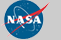Curator: David M. Giles NASA Official: Brent N. Holben Generated: 12/12/2019